# Frank Solutions for Class 9 Maths Chapter 8 Simultaneous Linear Equations

Frank Solutions for Class 9 Maths Chapter 8 Simultaneous Linear Equations has precise answers to help students in their exam preparation. These solutions are the best study resource in clearing student’s doubts quickly. Practising Frank Solutions on a daily basis improves students’ problem solving and time management skills, which are vital from an exam point of view. For securing more marks, students can take help of Frank Solutions for Class 9 Maths Chapter 8 Simultaneous Linear Equations PDF, from the links which are given below

Chapter 8 explains the problems based on linear equations with examples. The solutions are formulated by expert teachers, with the aim of helping students, to boost confidence in a subject like Mathematics. These solutions are available 24 / 7, as per the student’s requirements.

## Frank Solutions for Class 9 Maths Chapter 8 Simultaneous Linear Equations Download PDF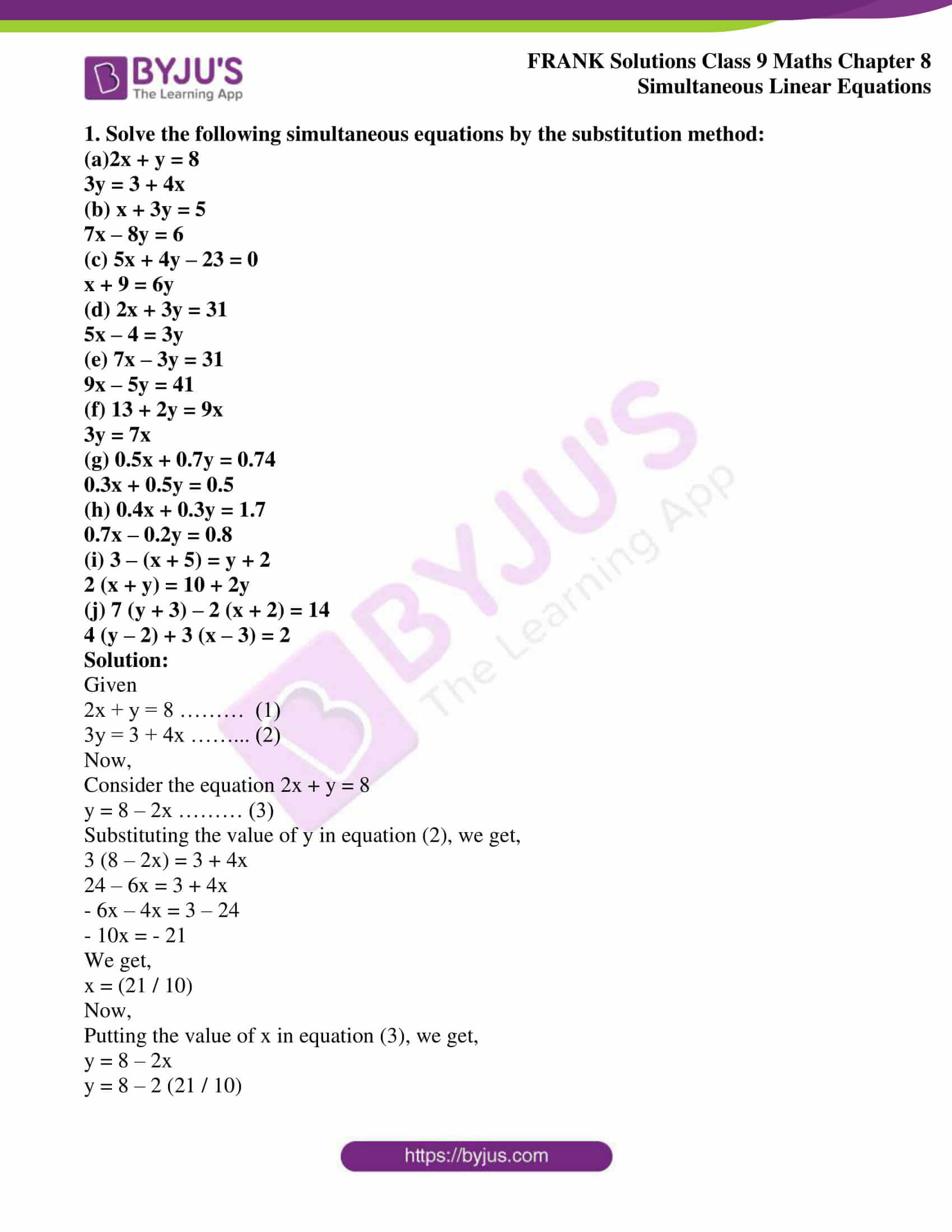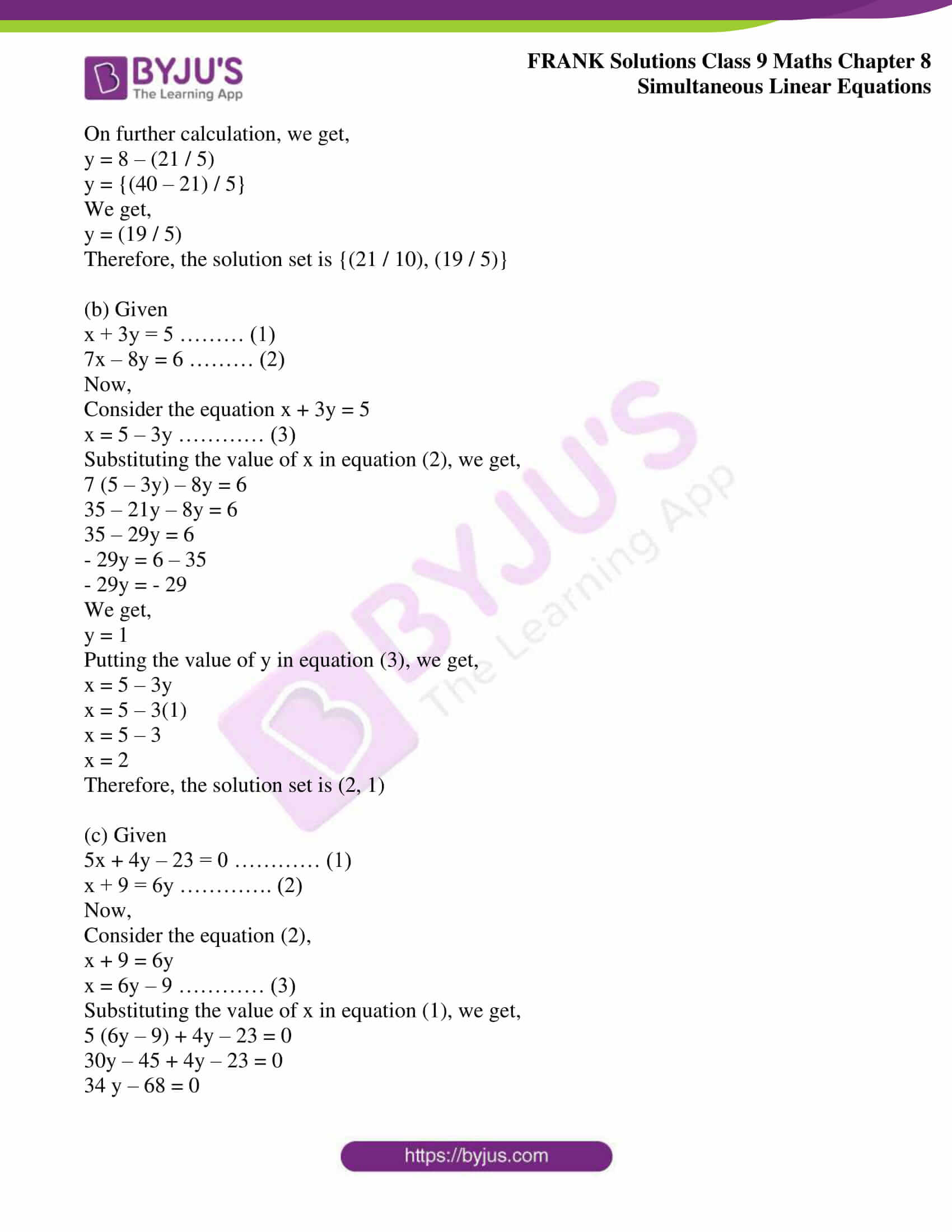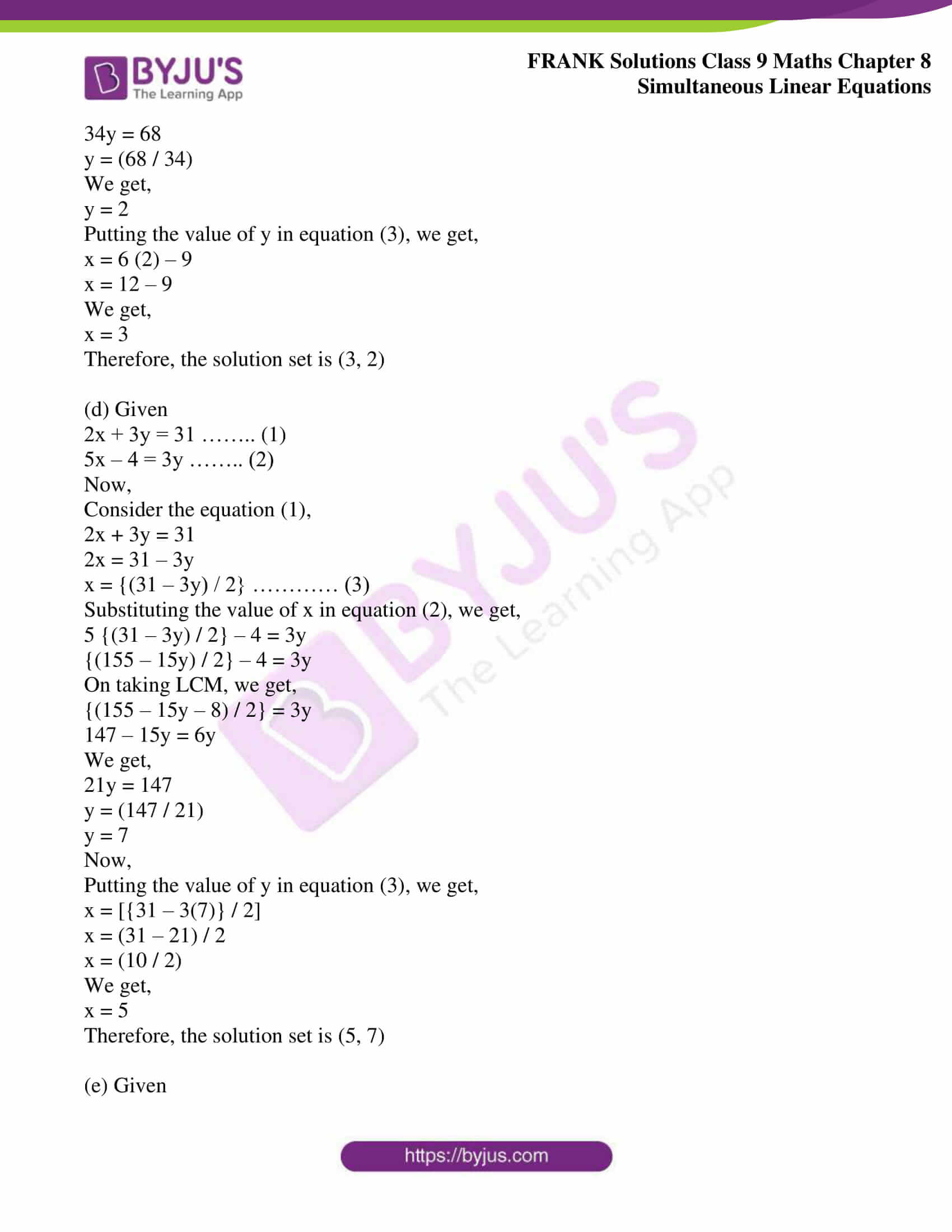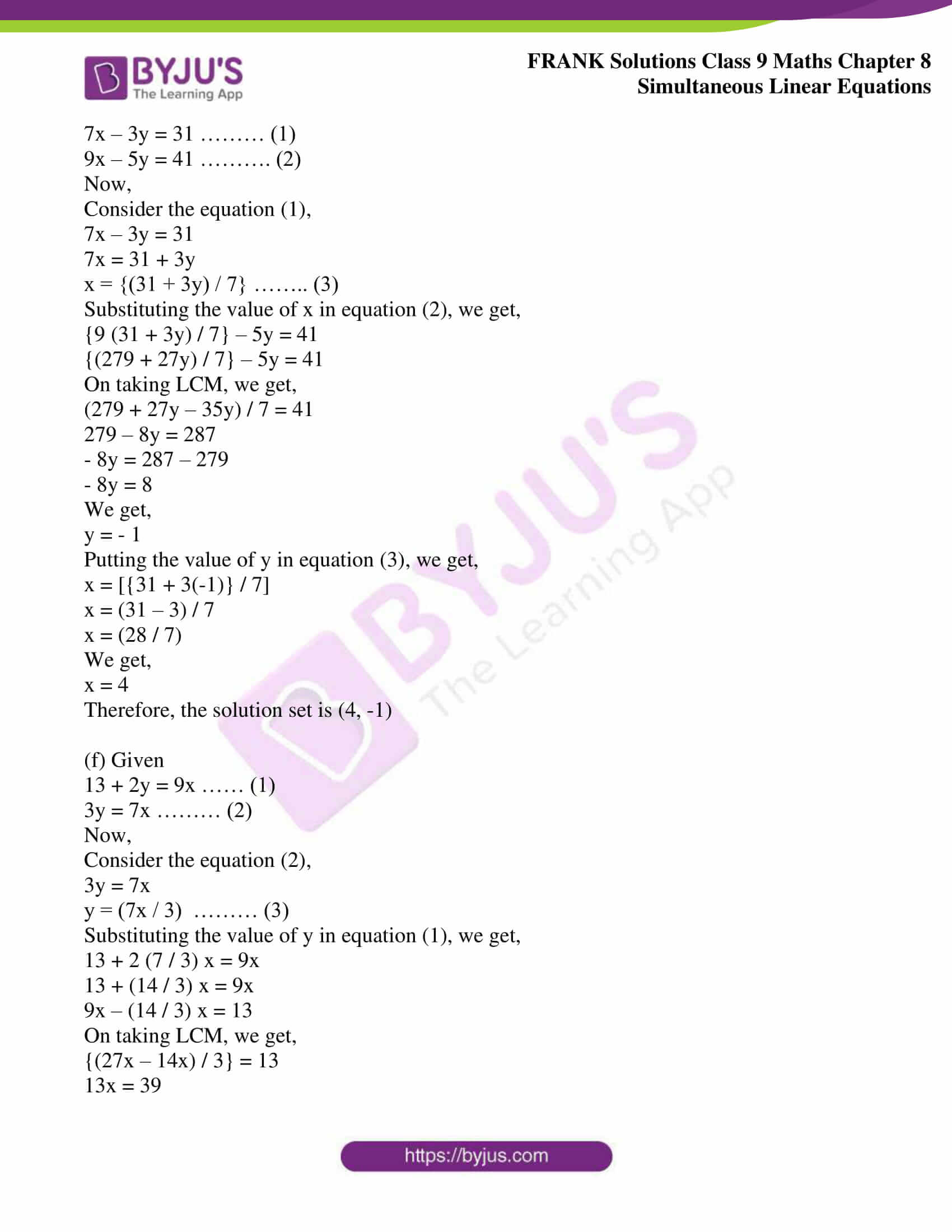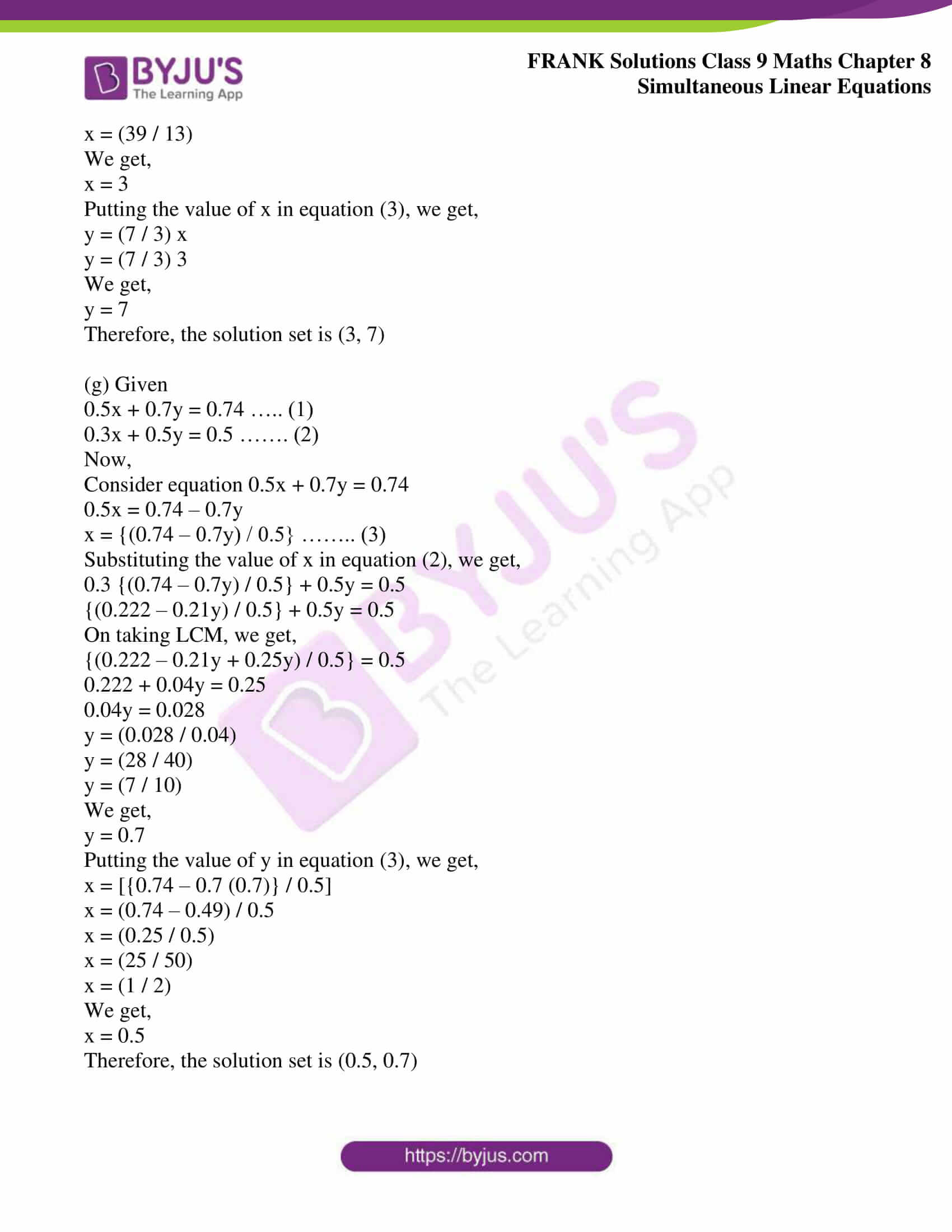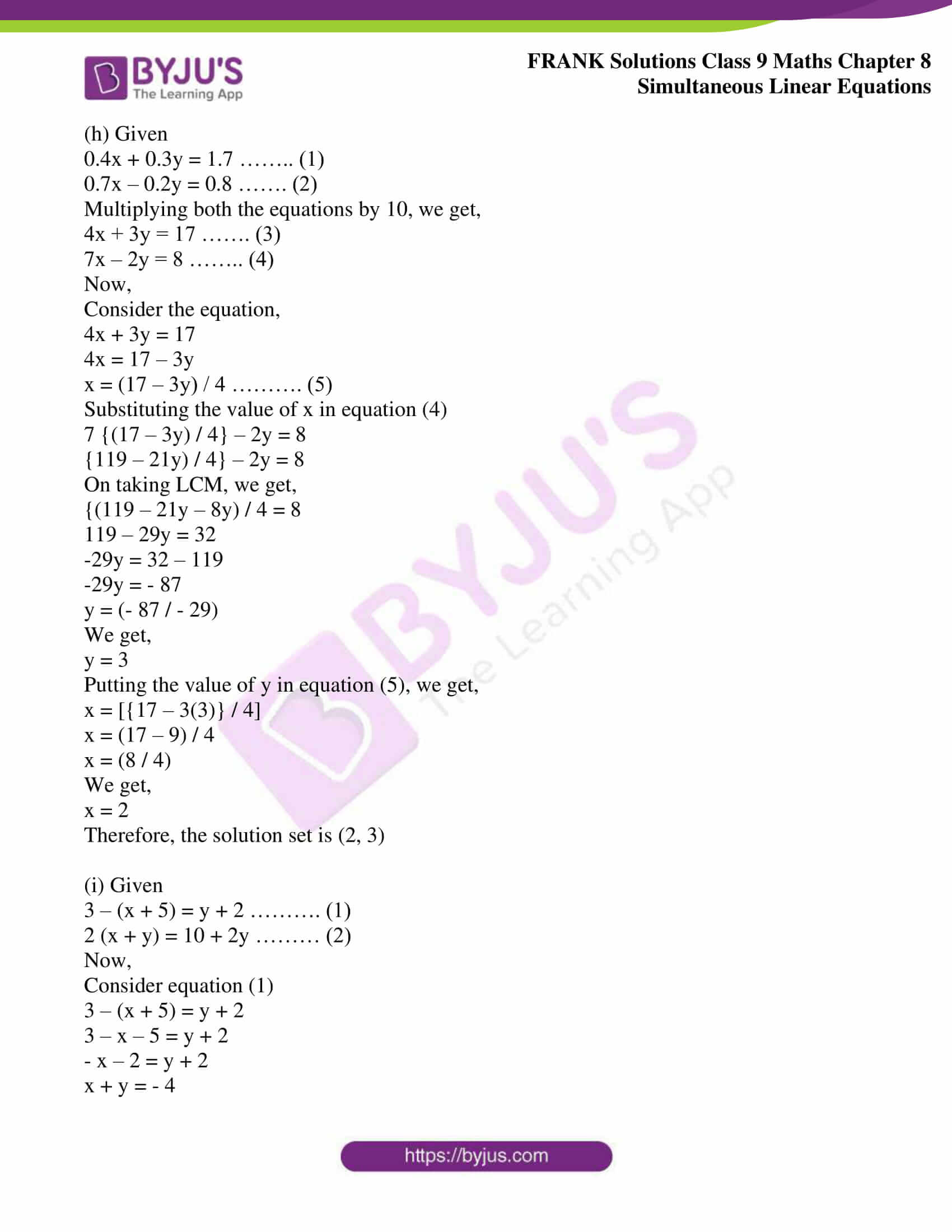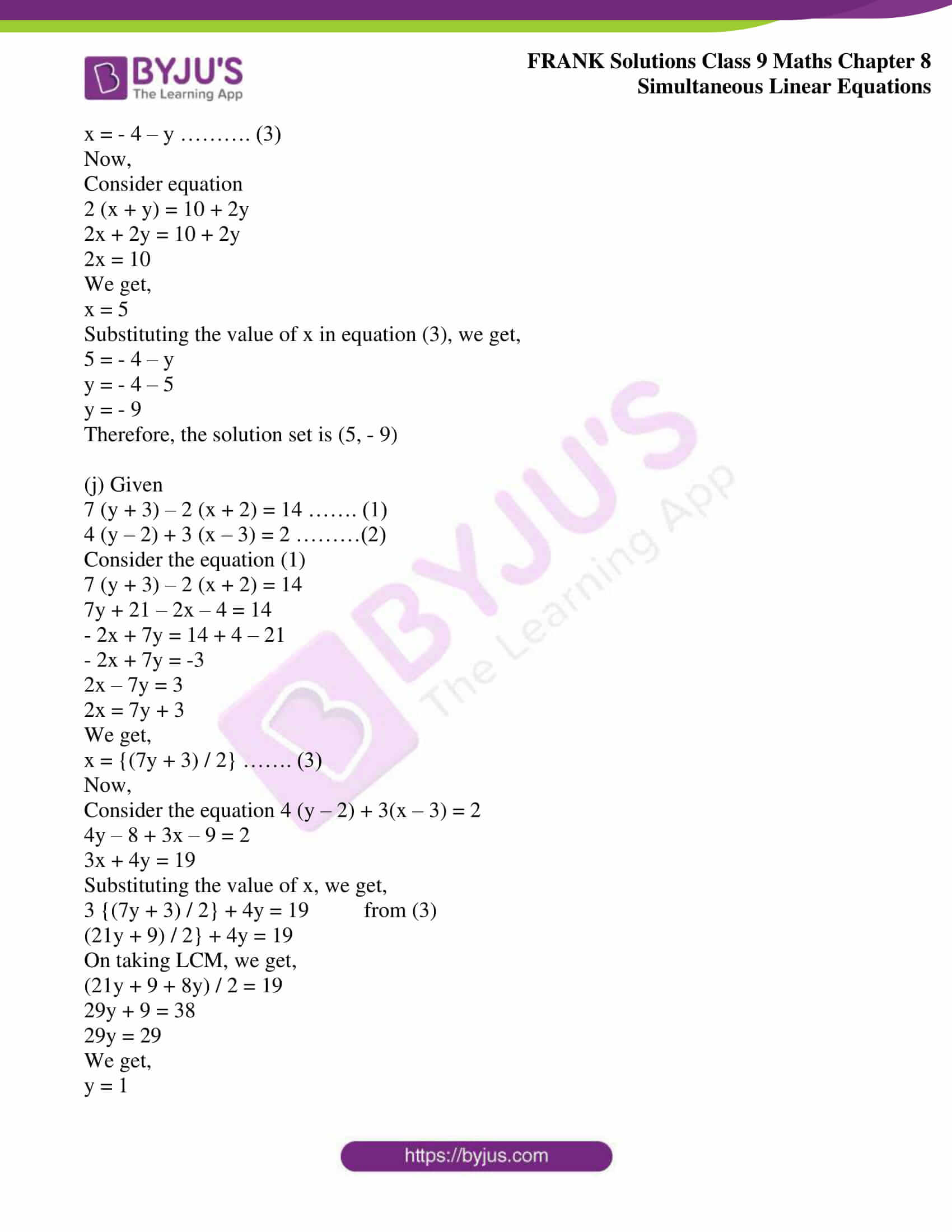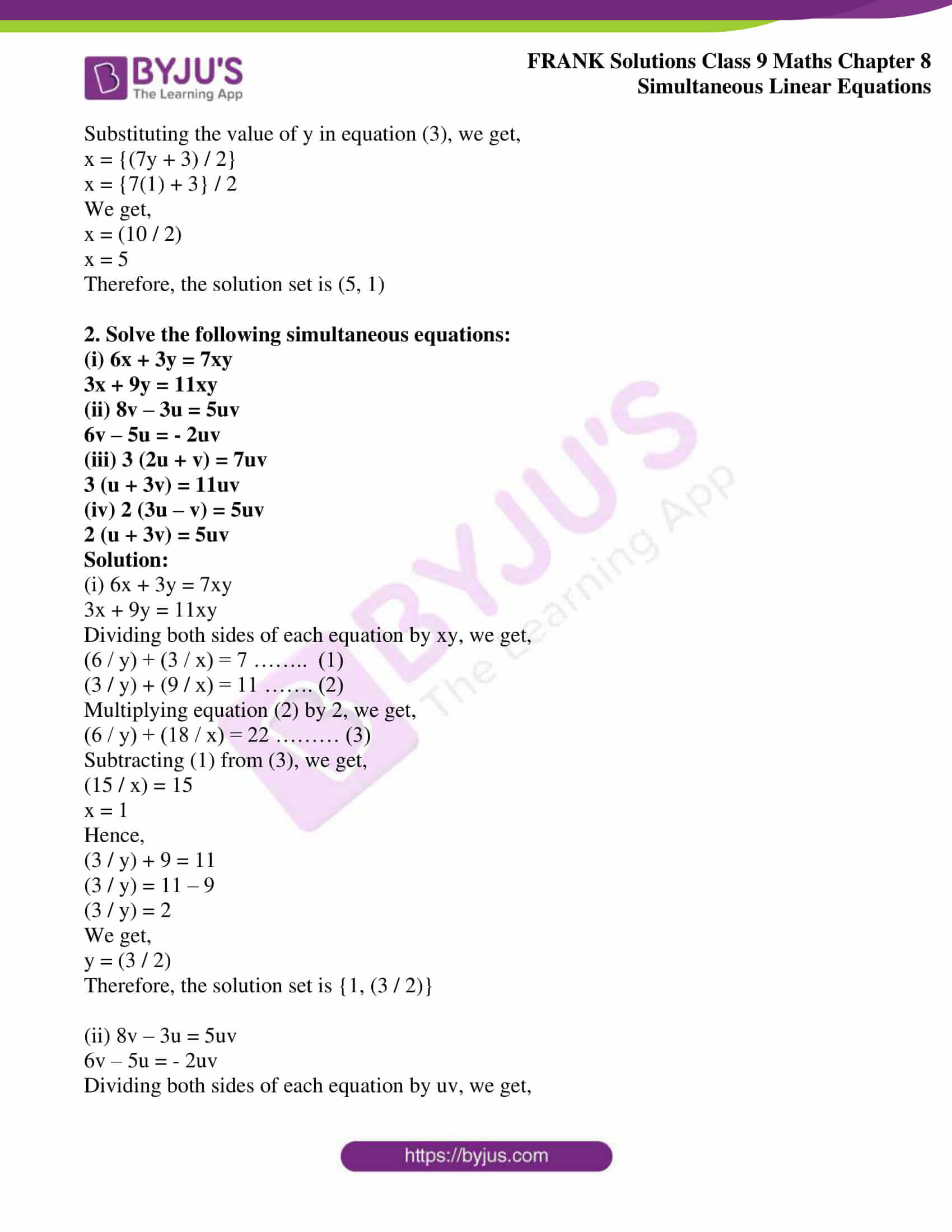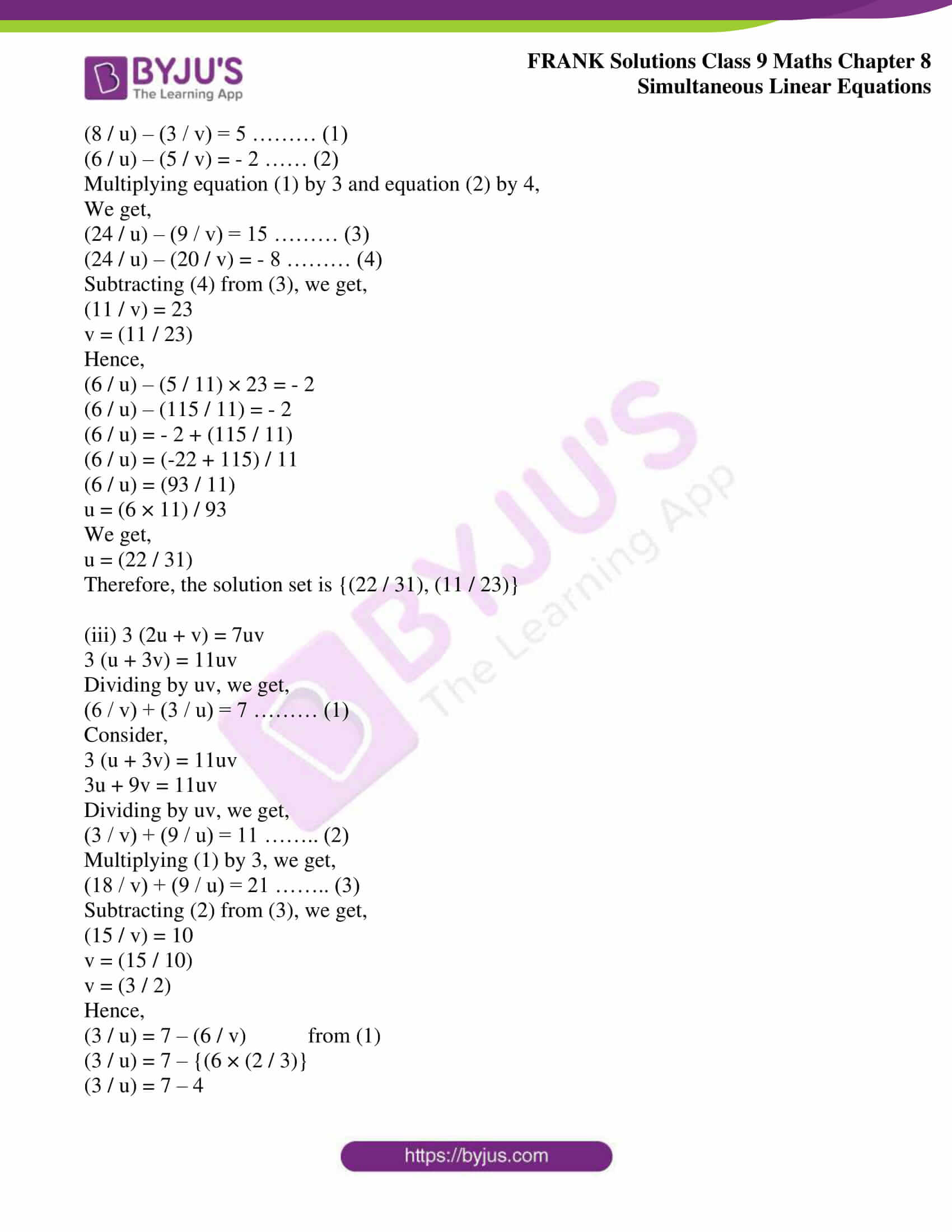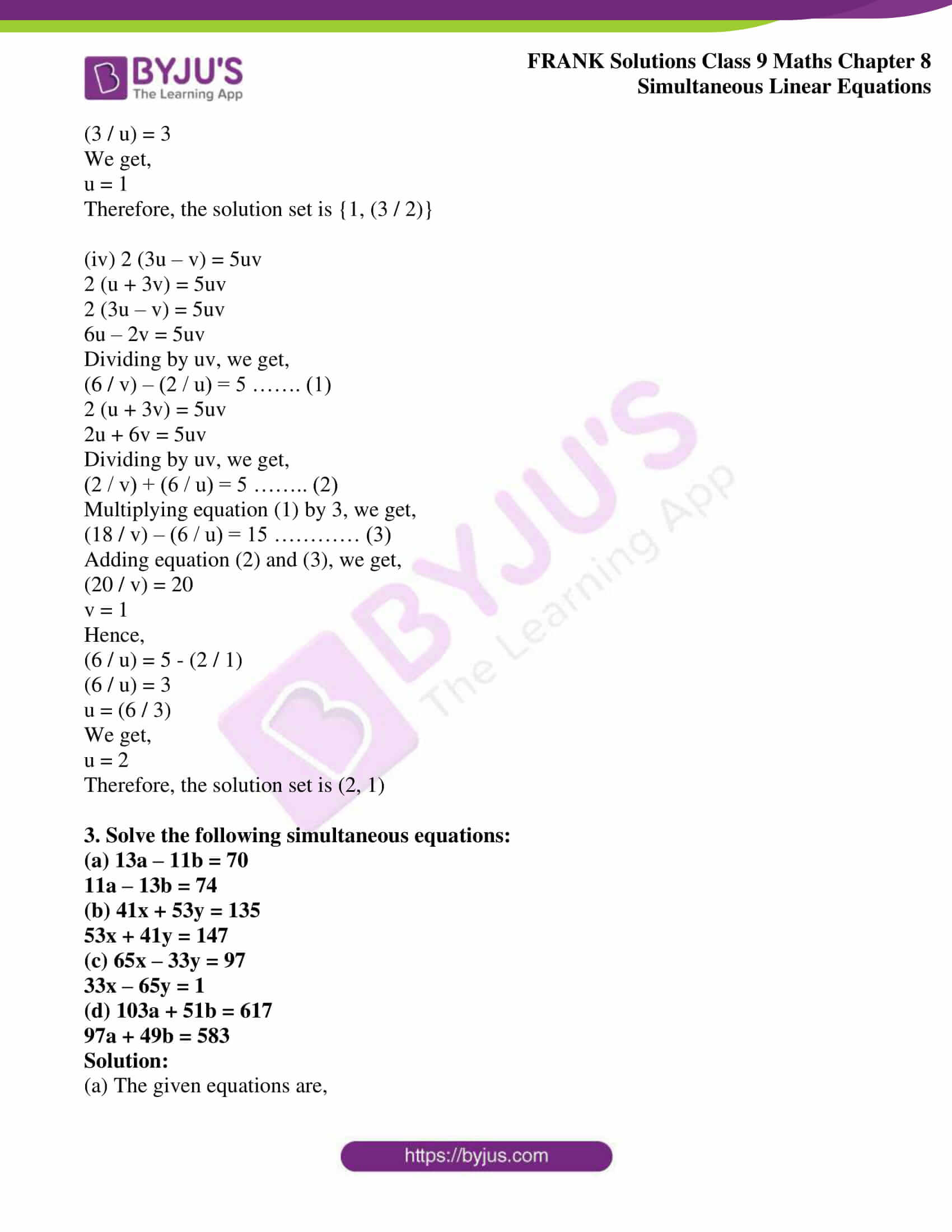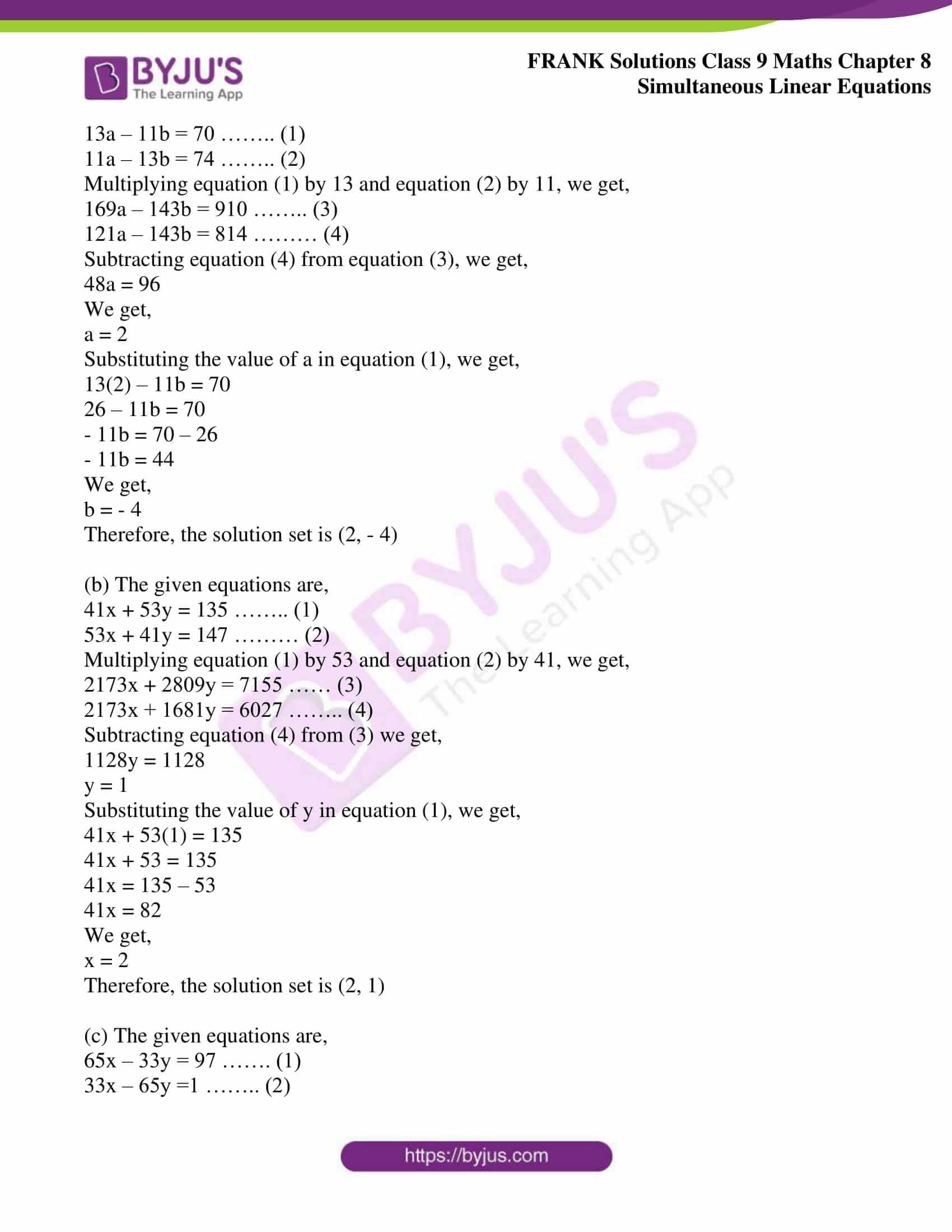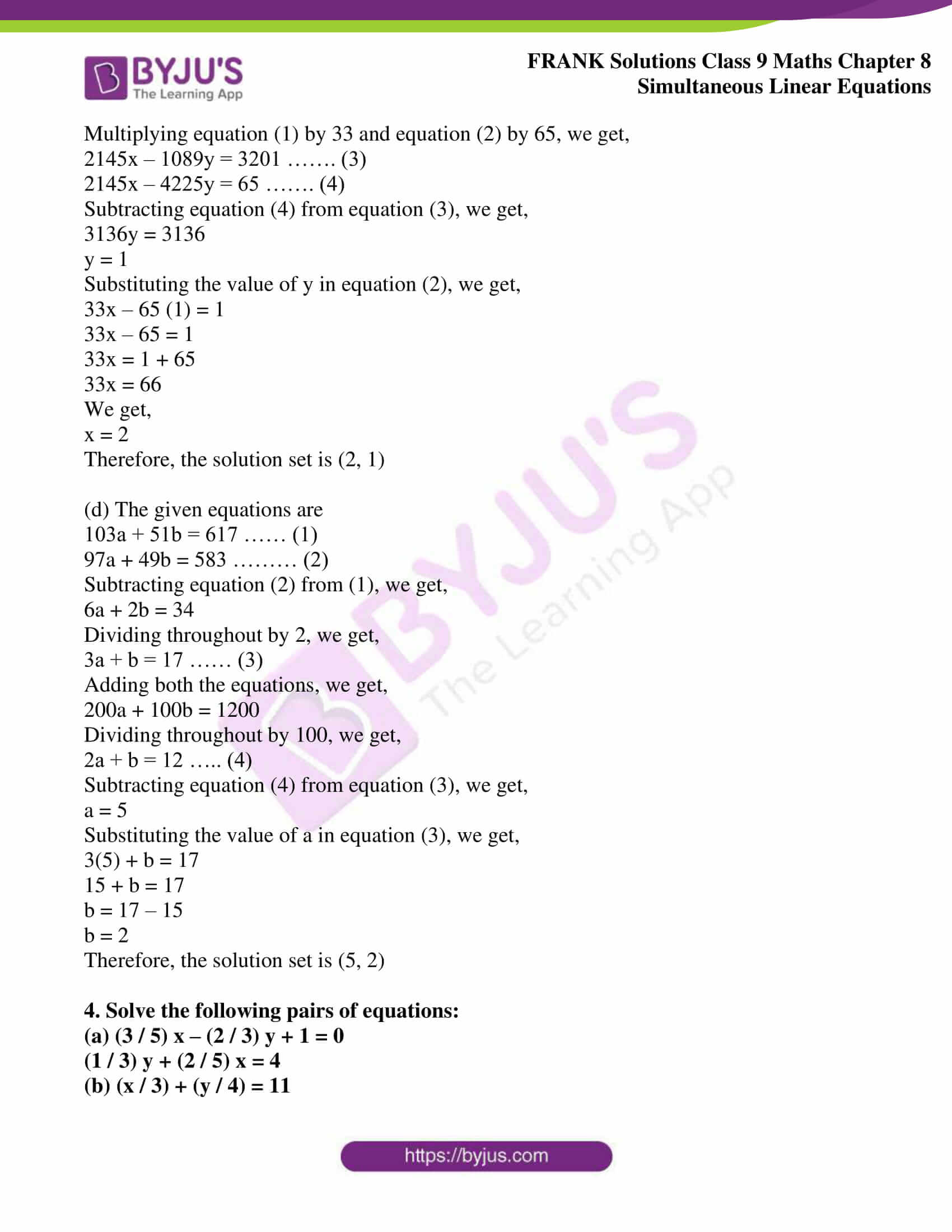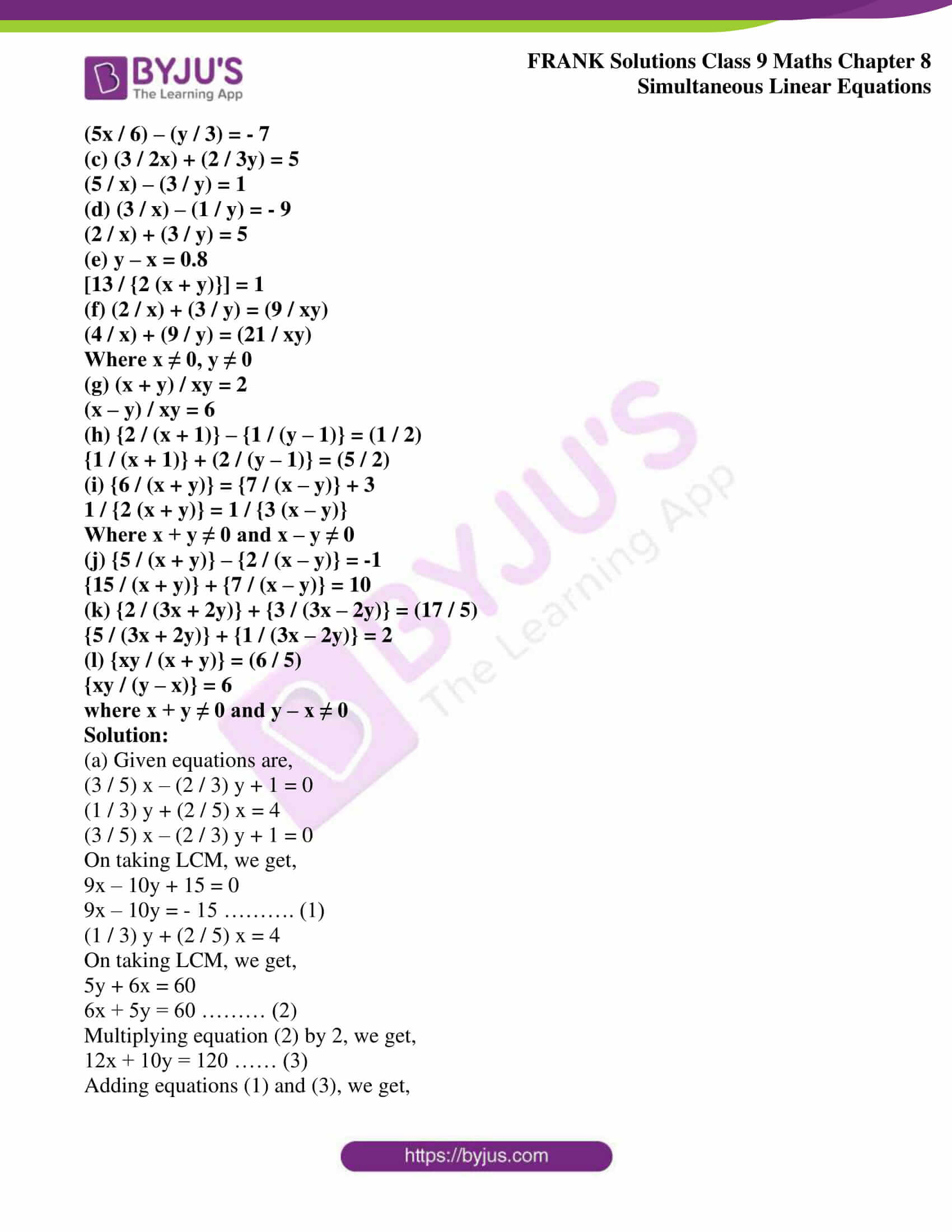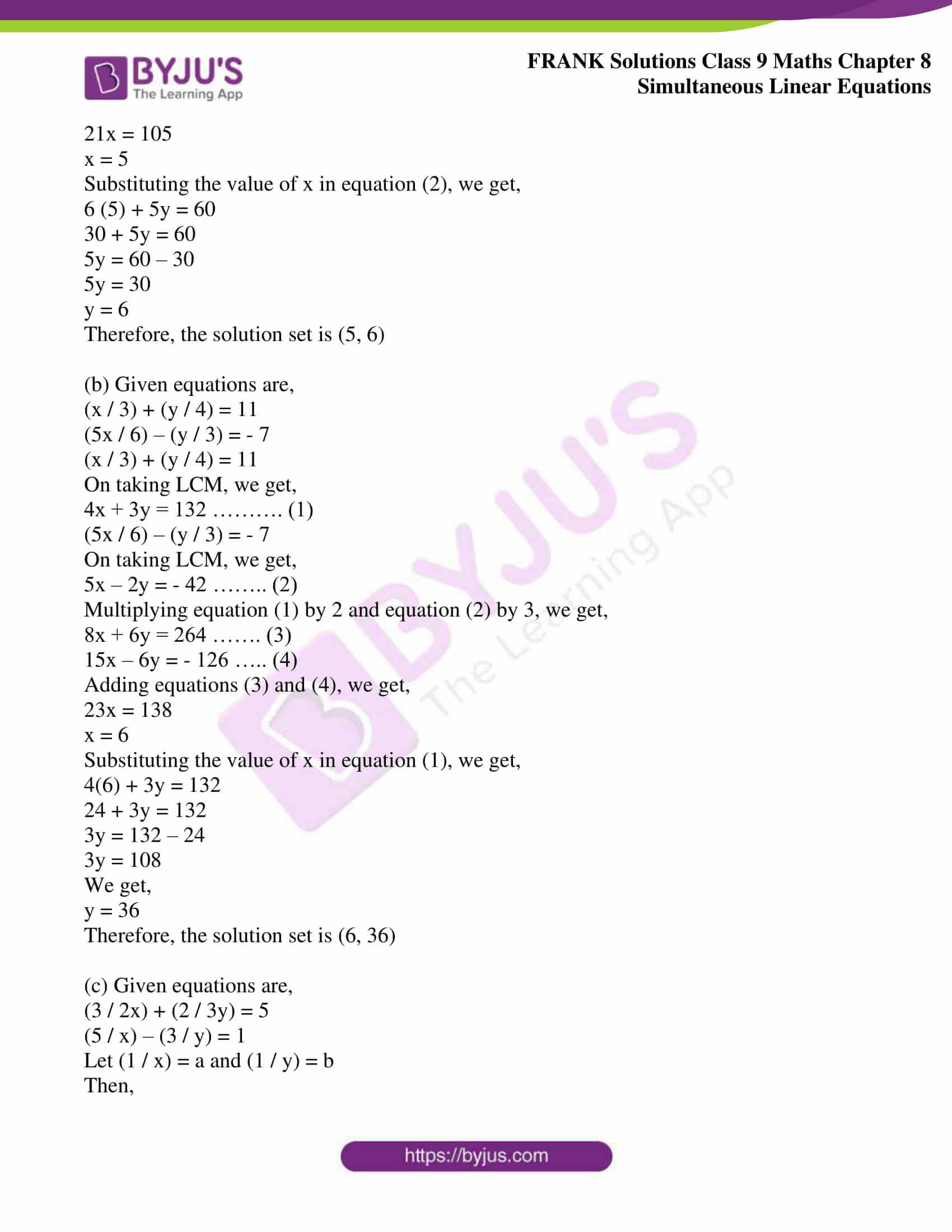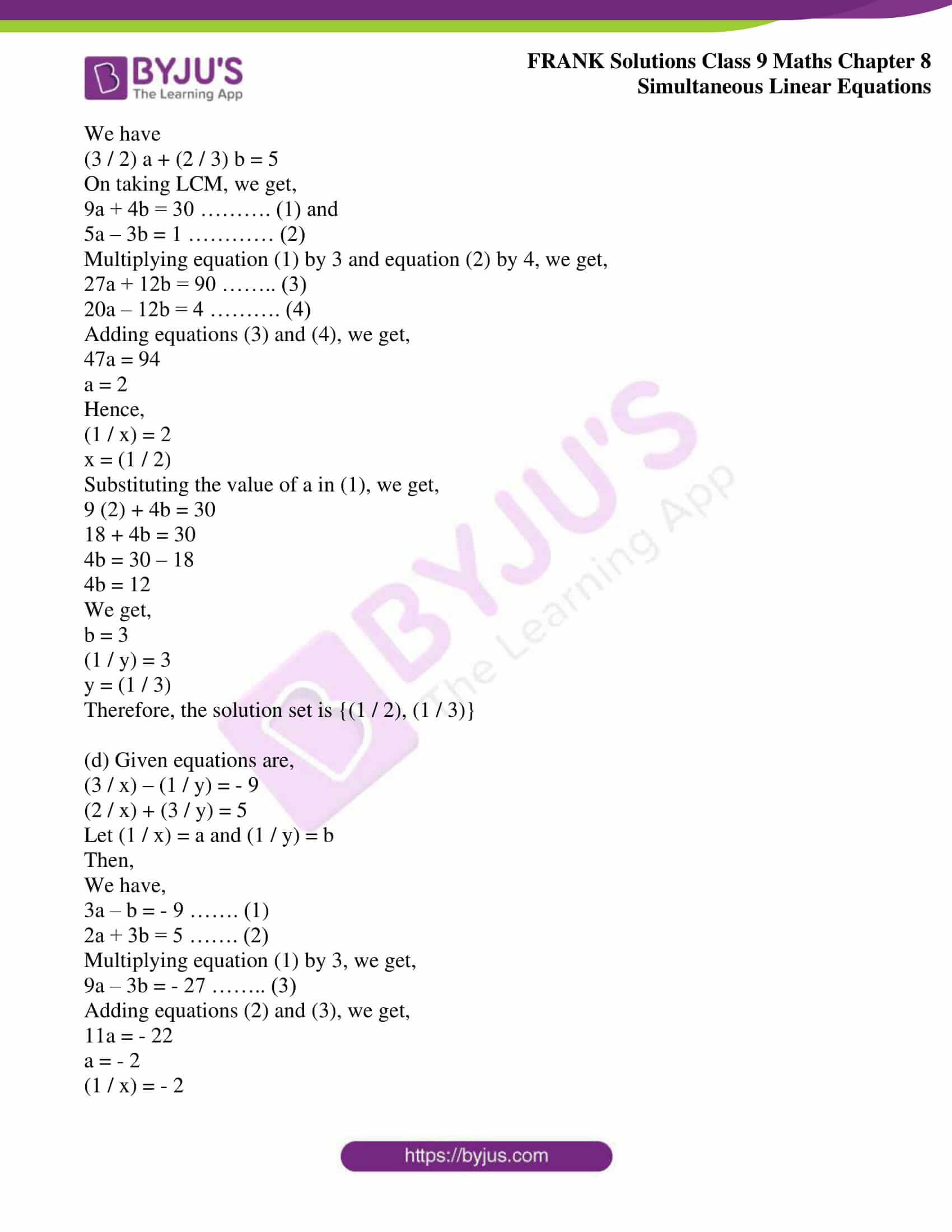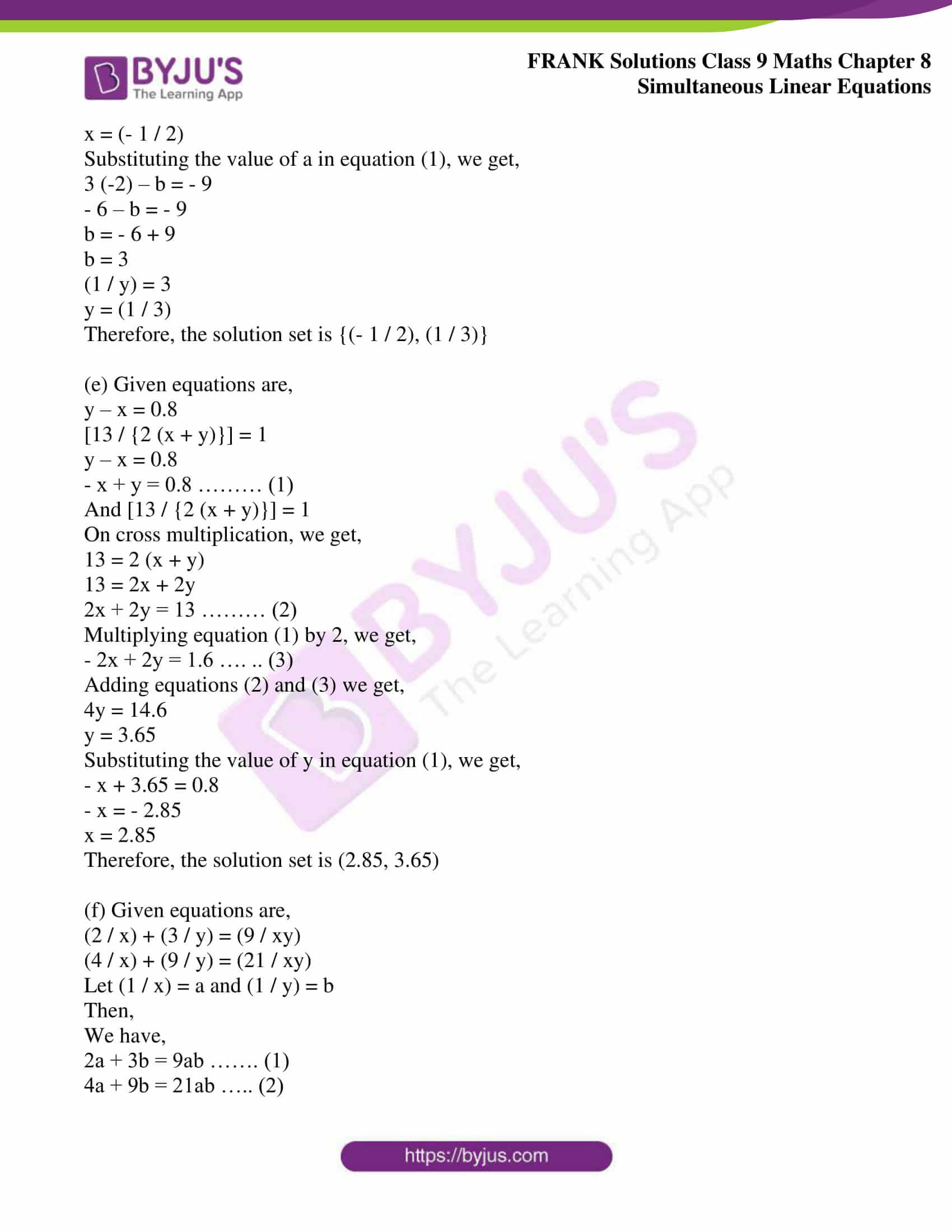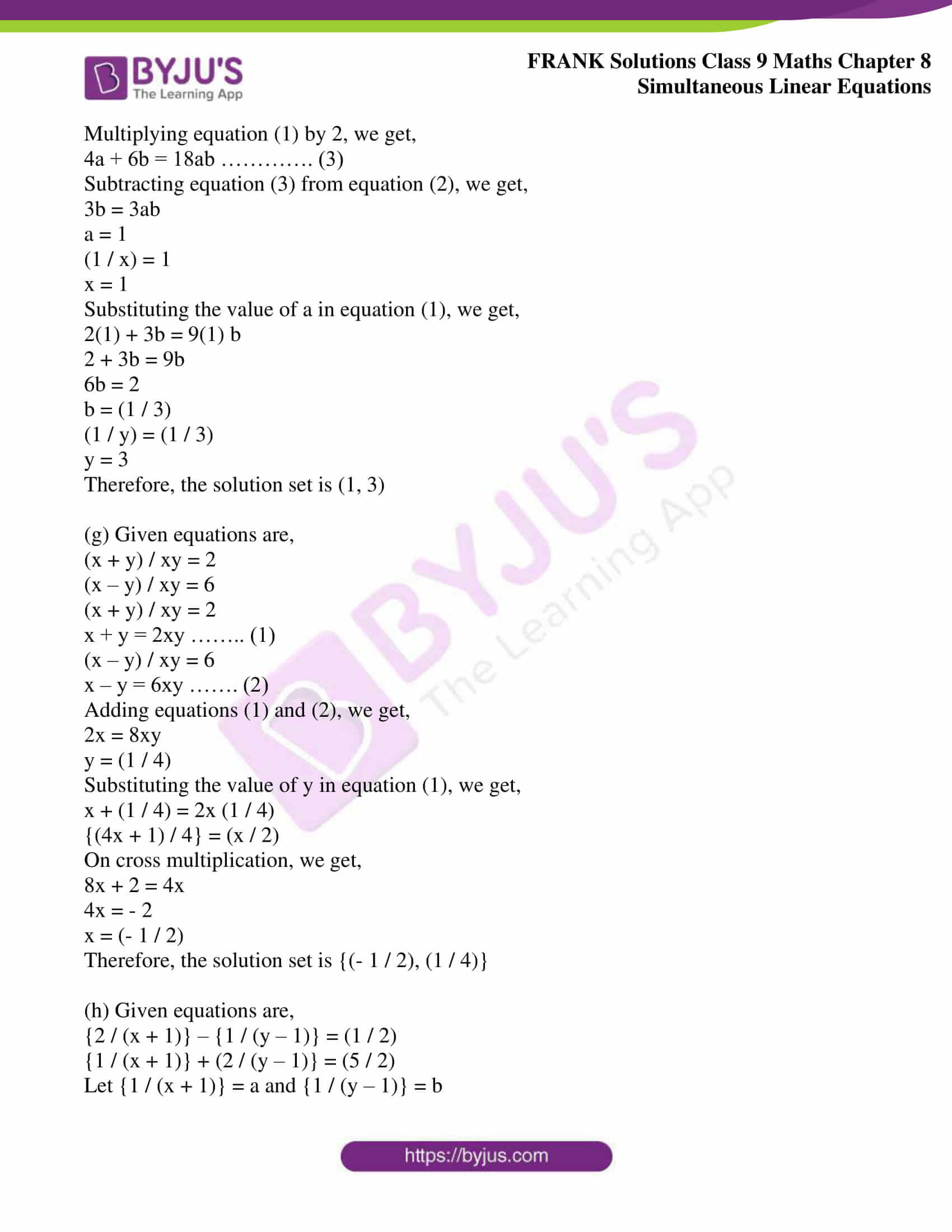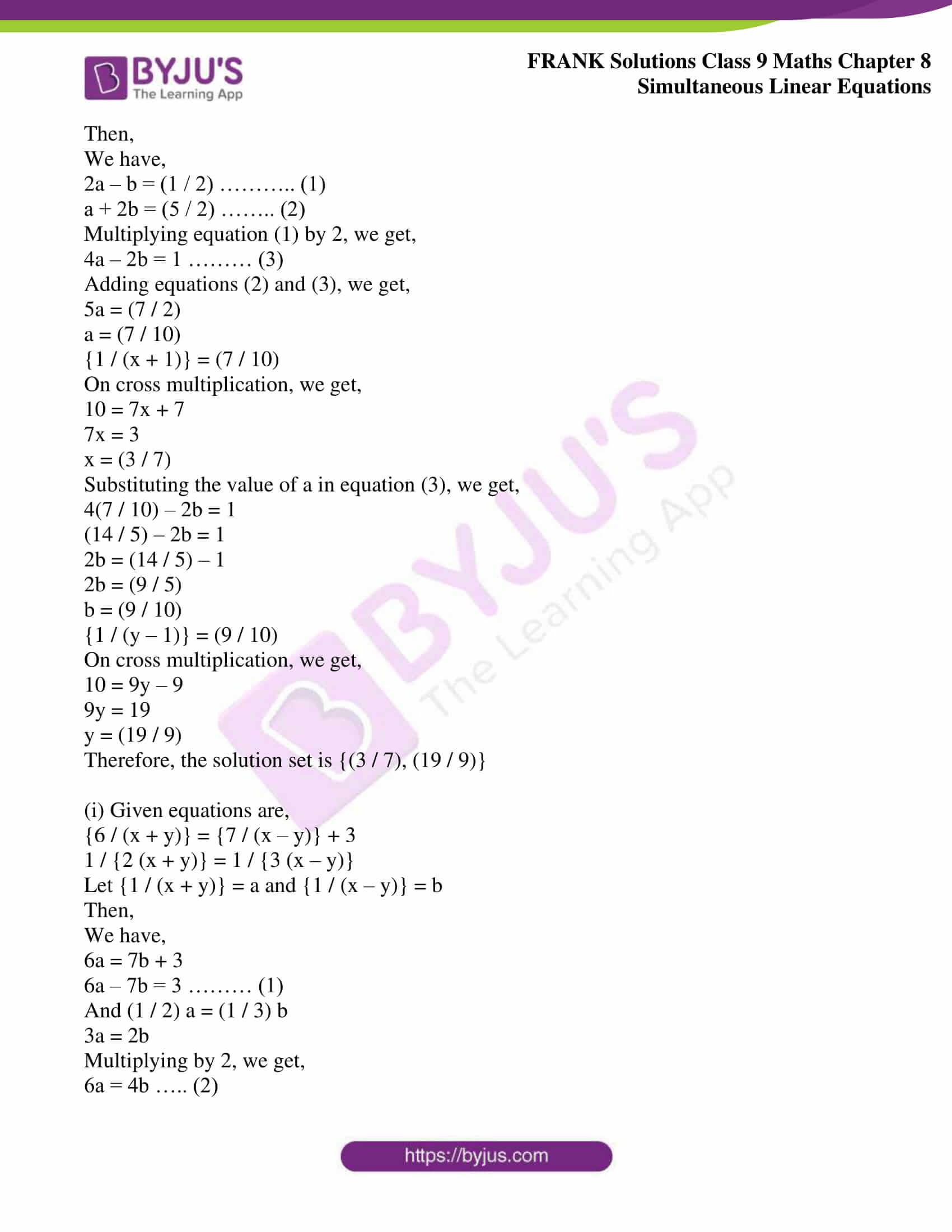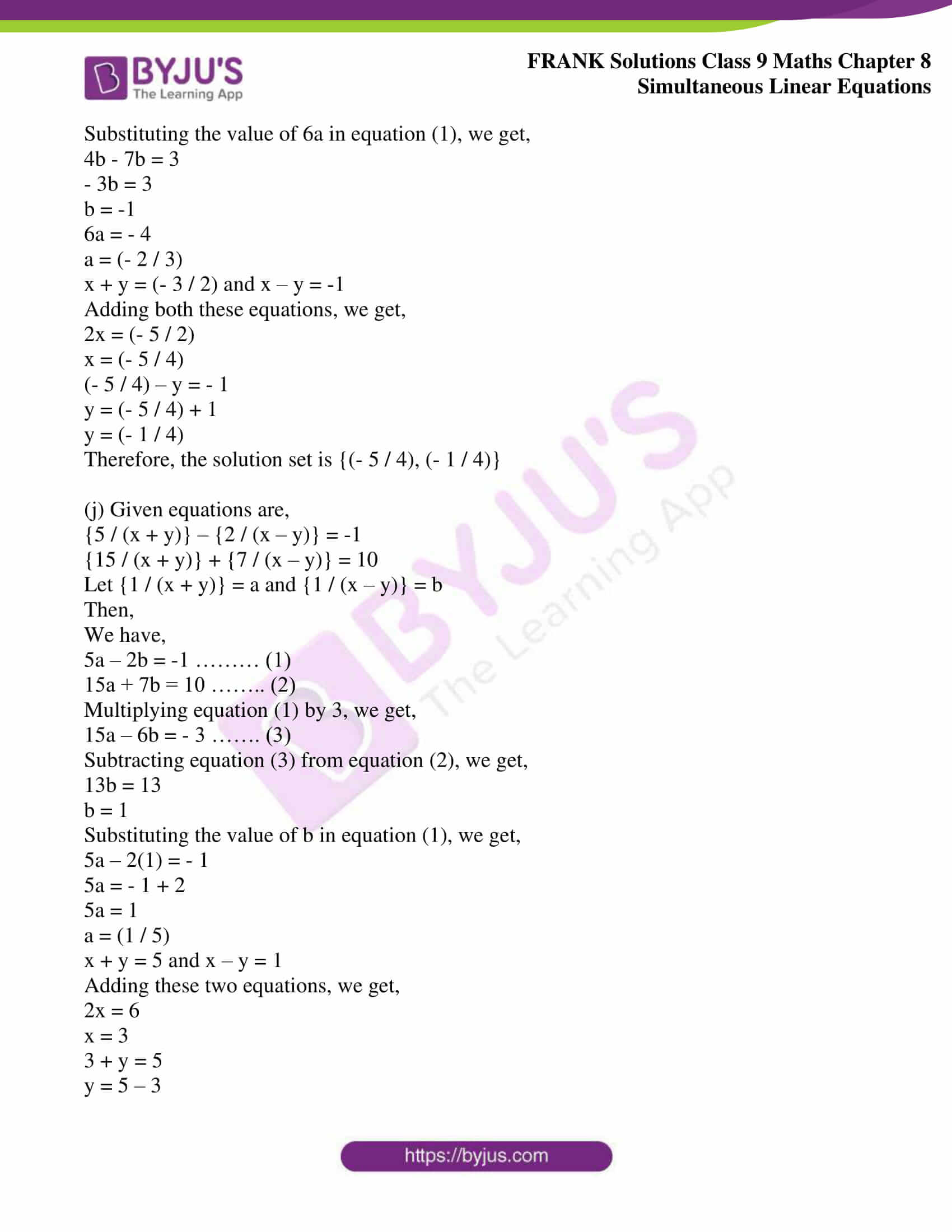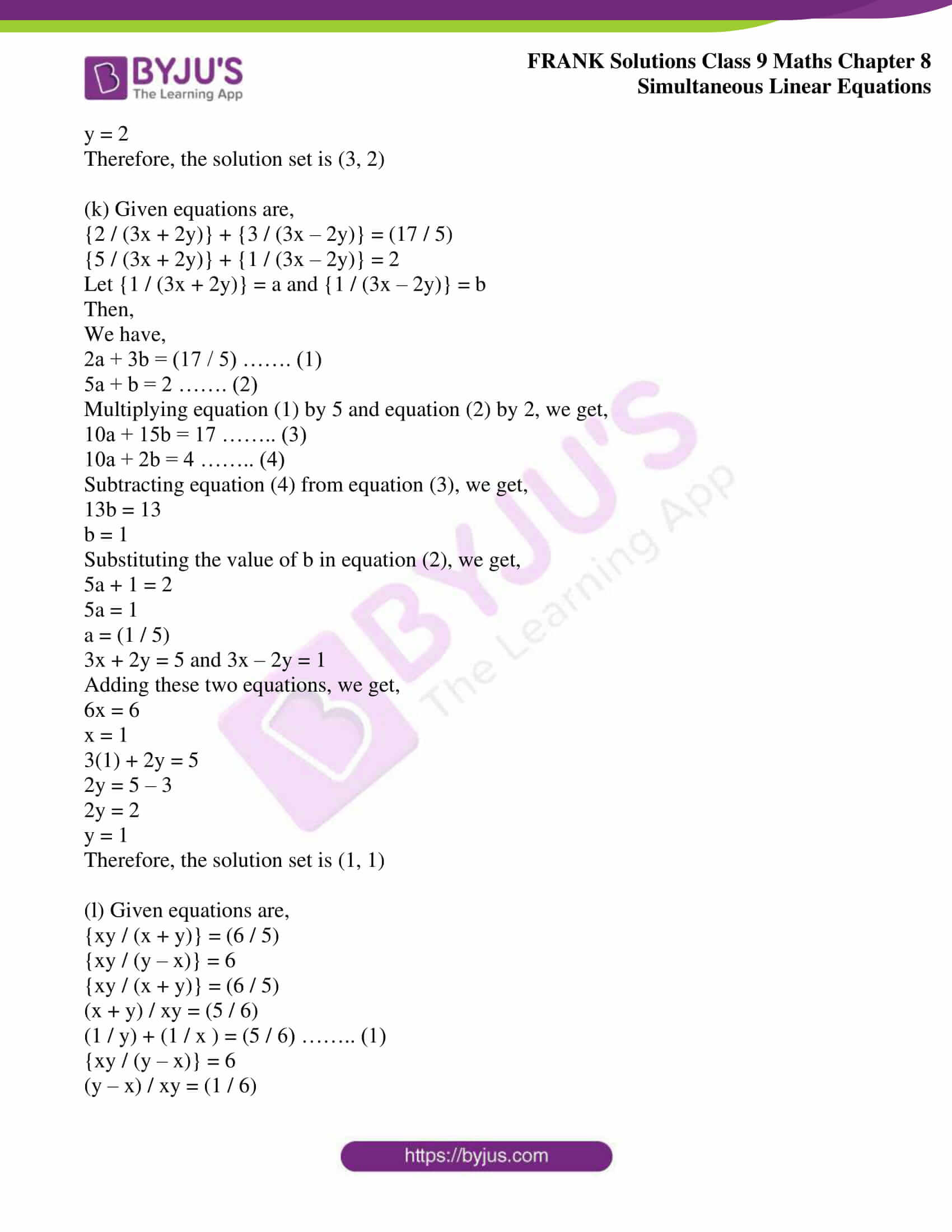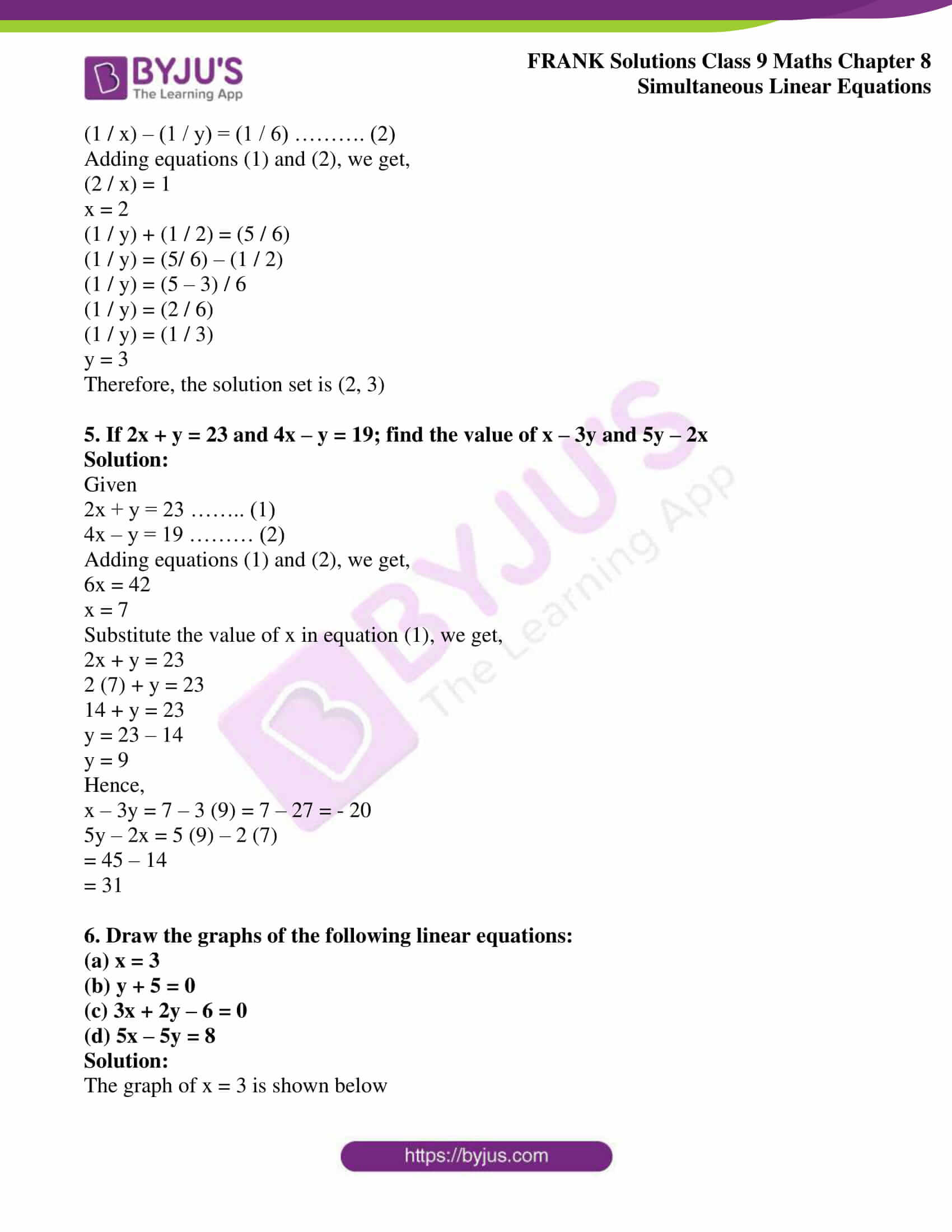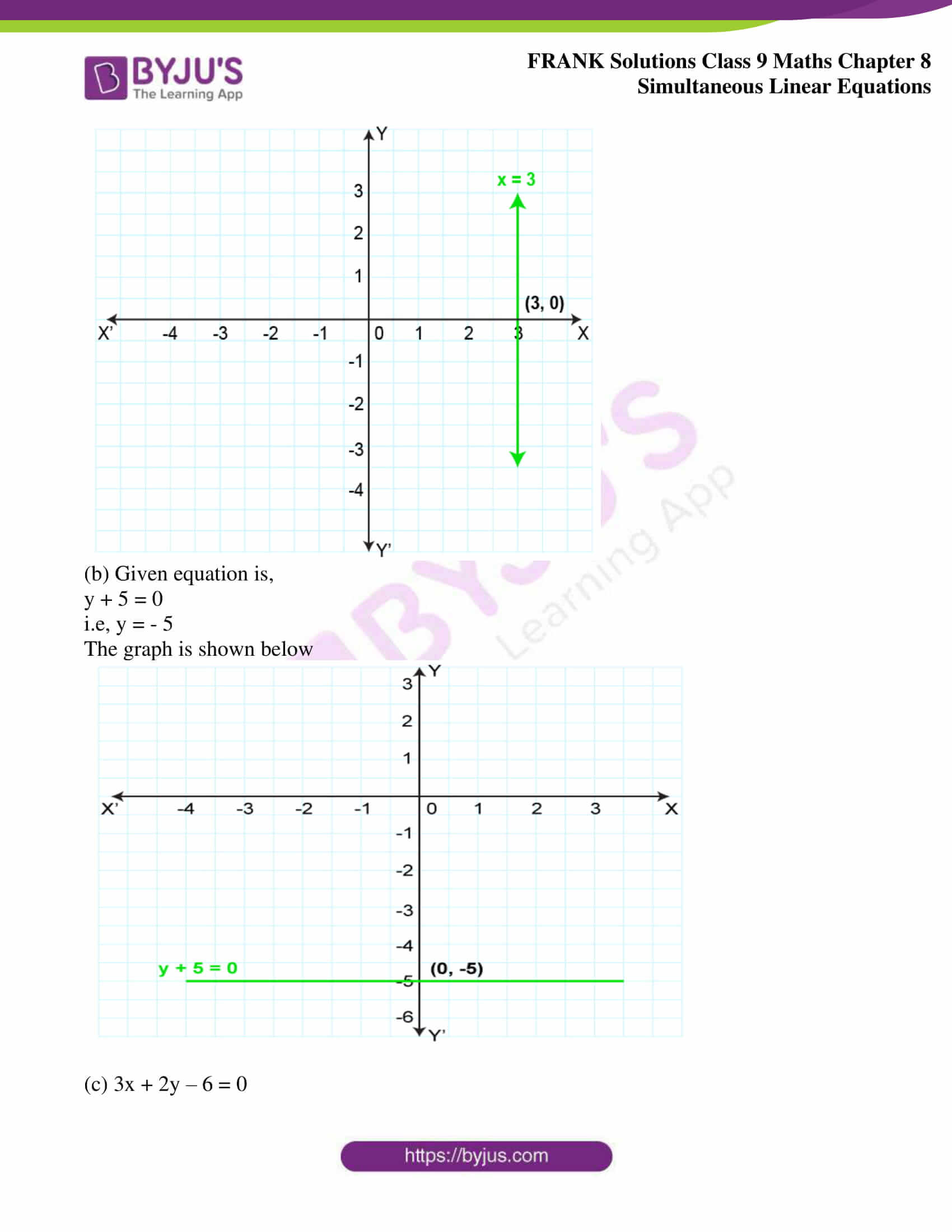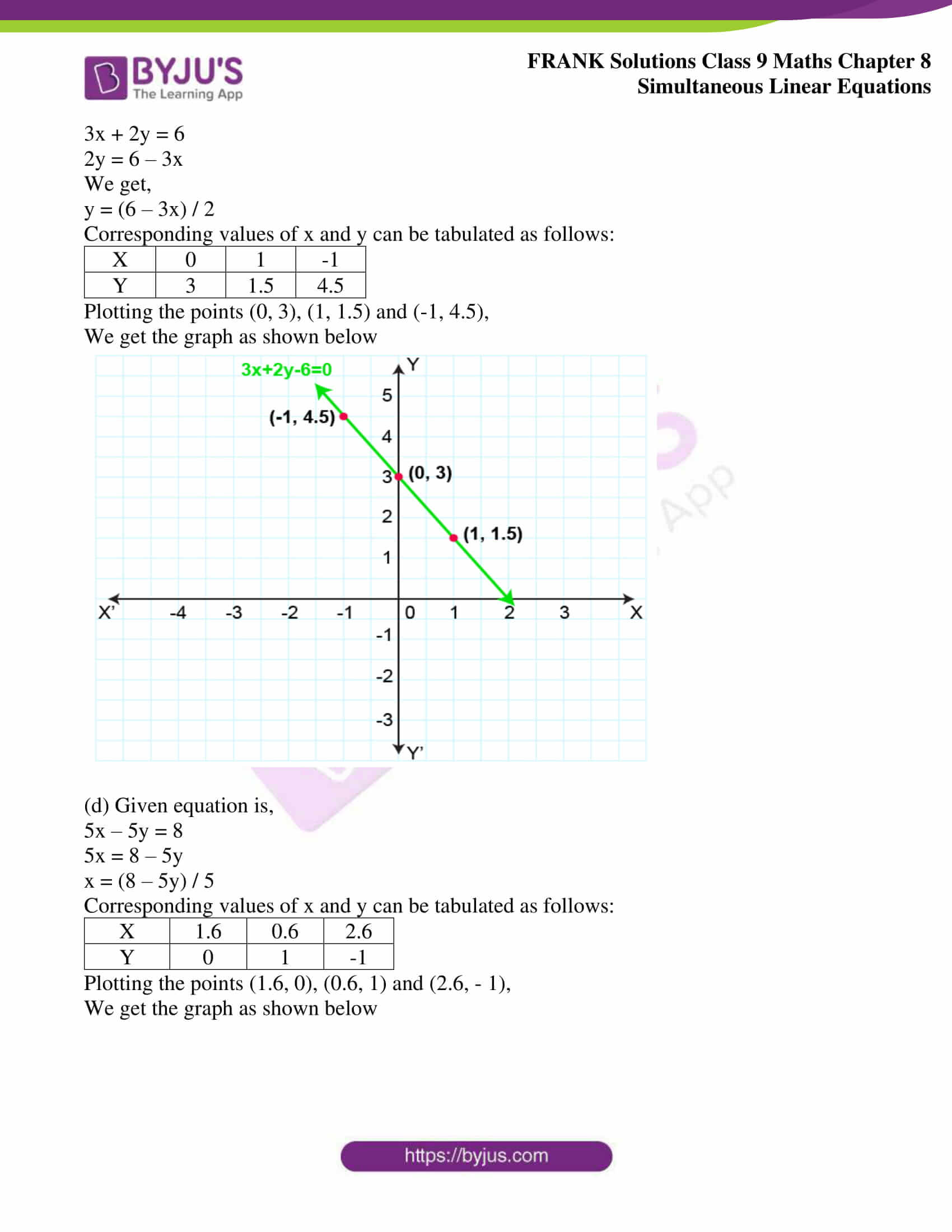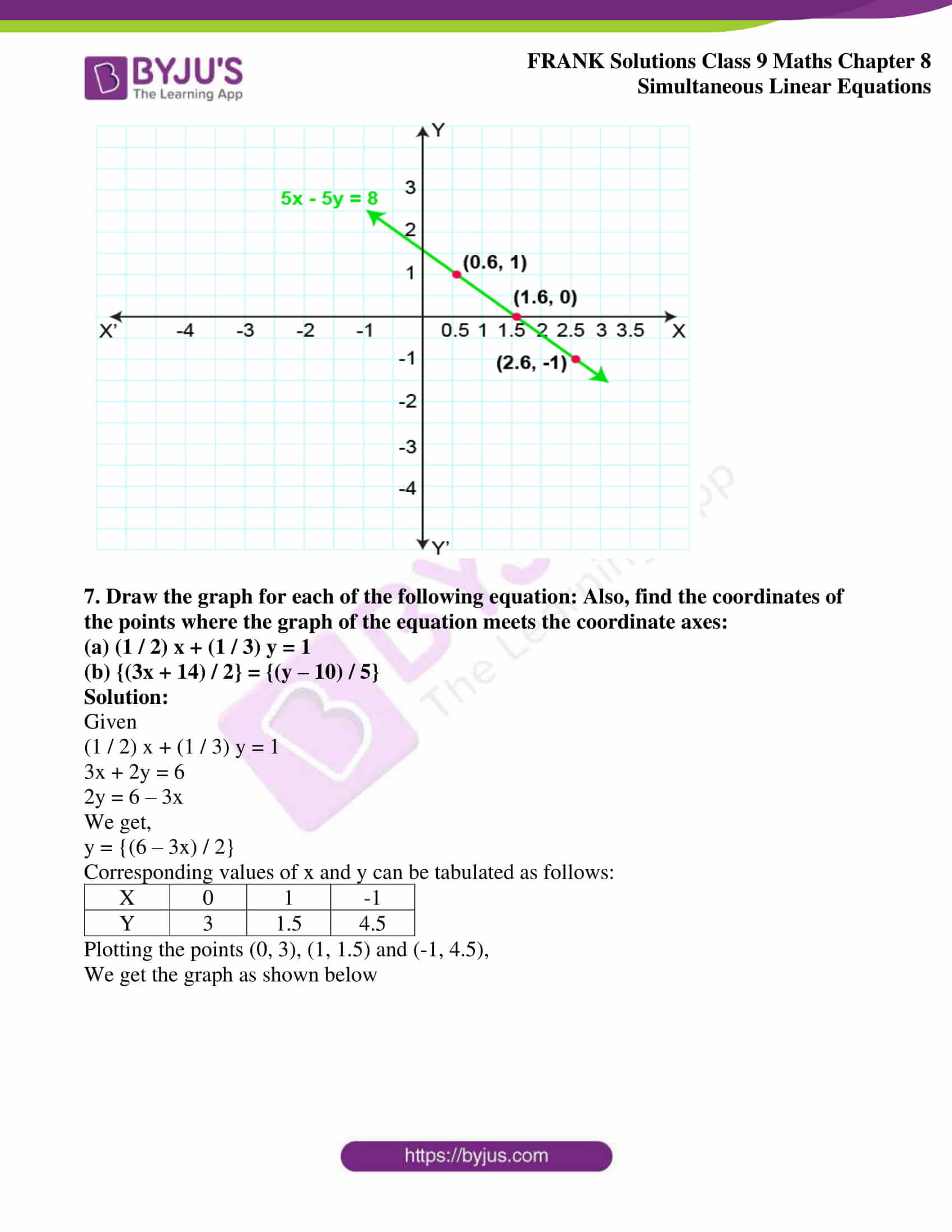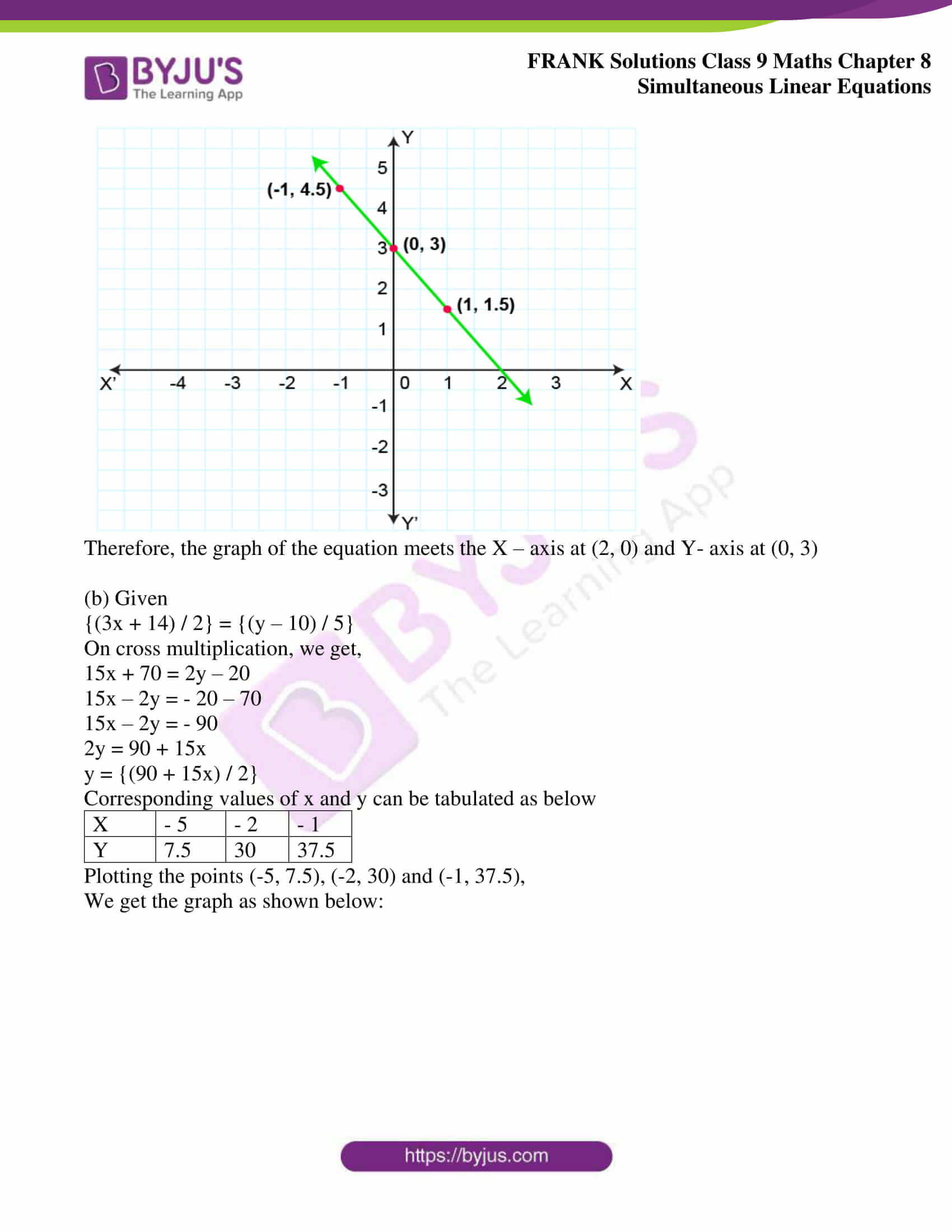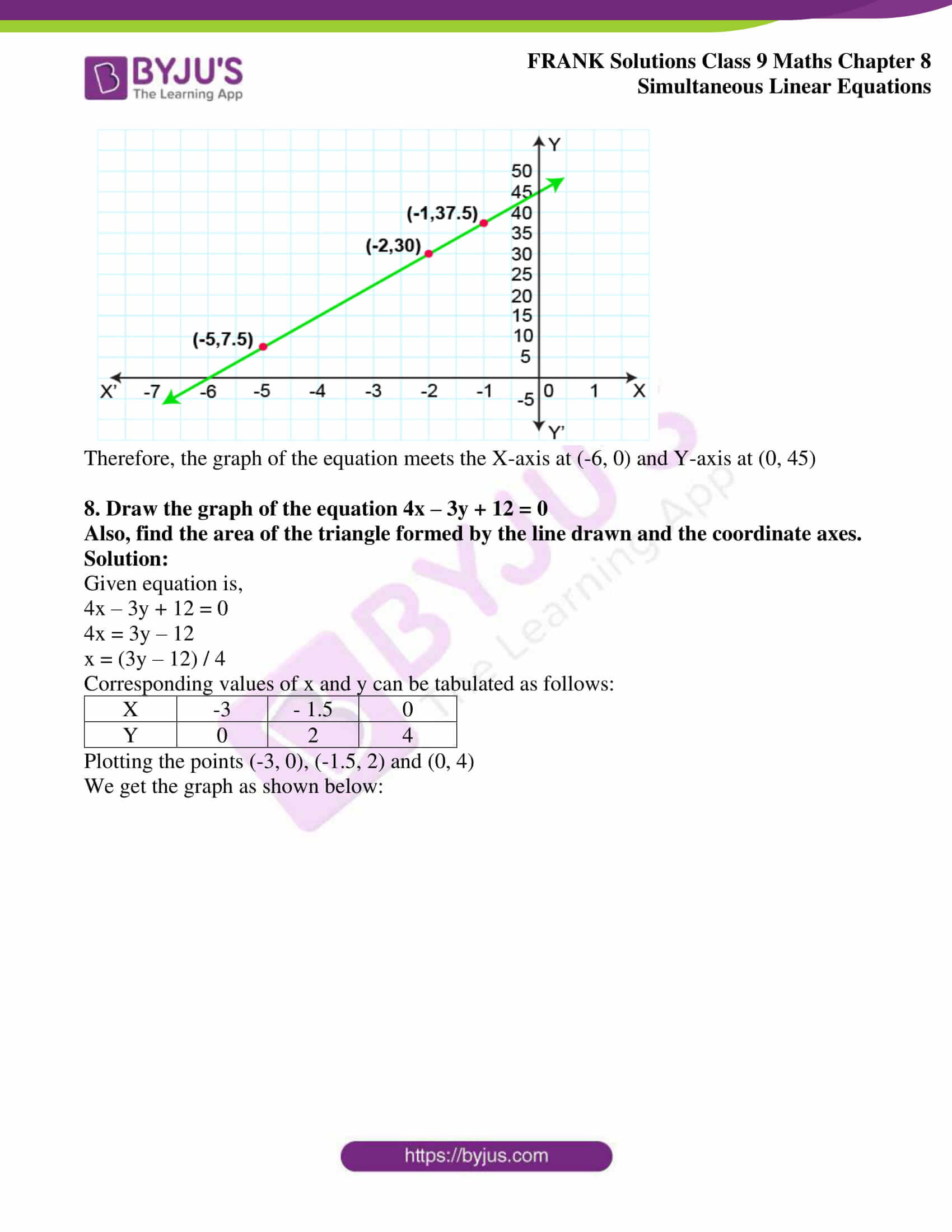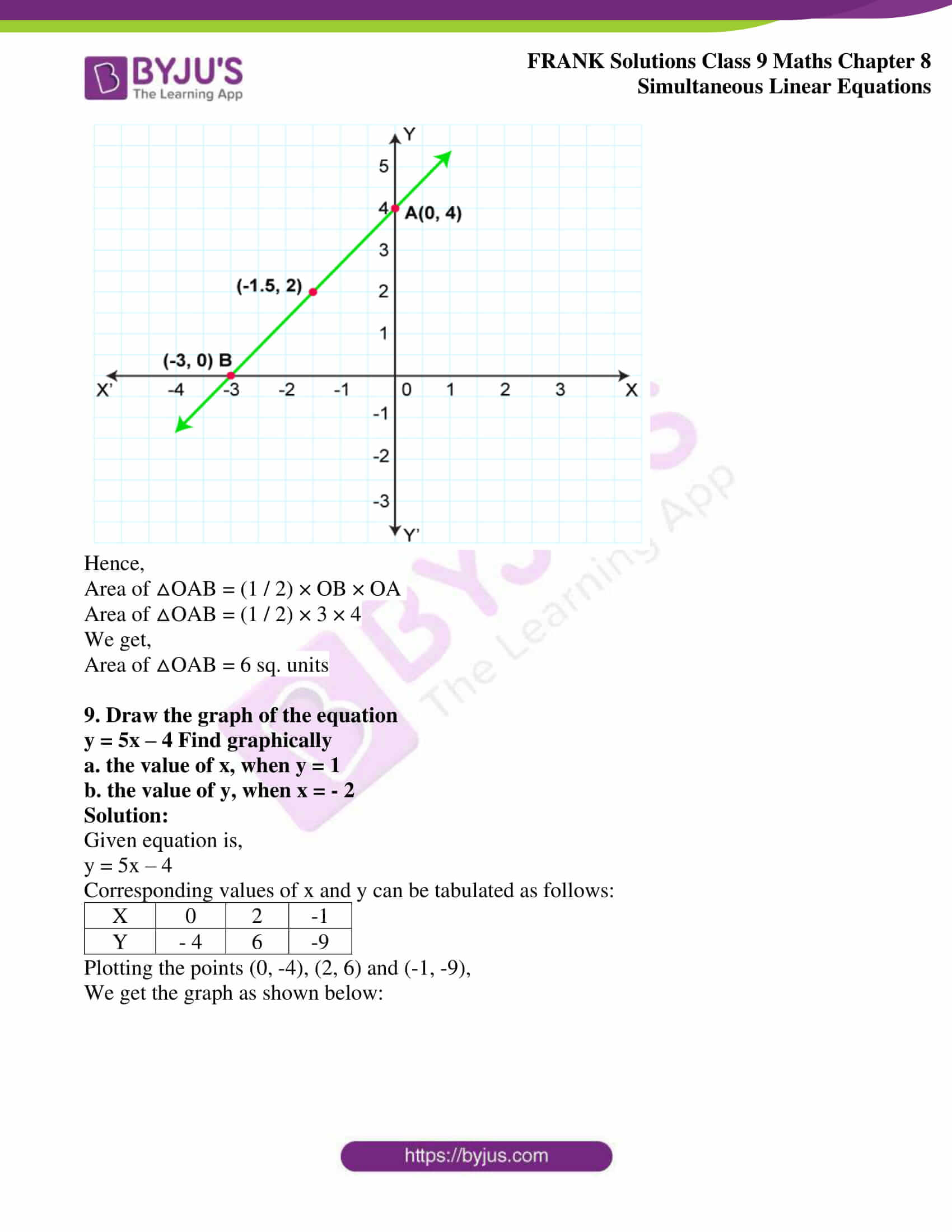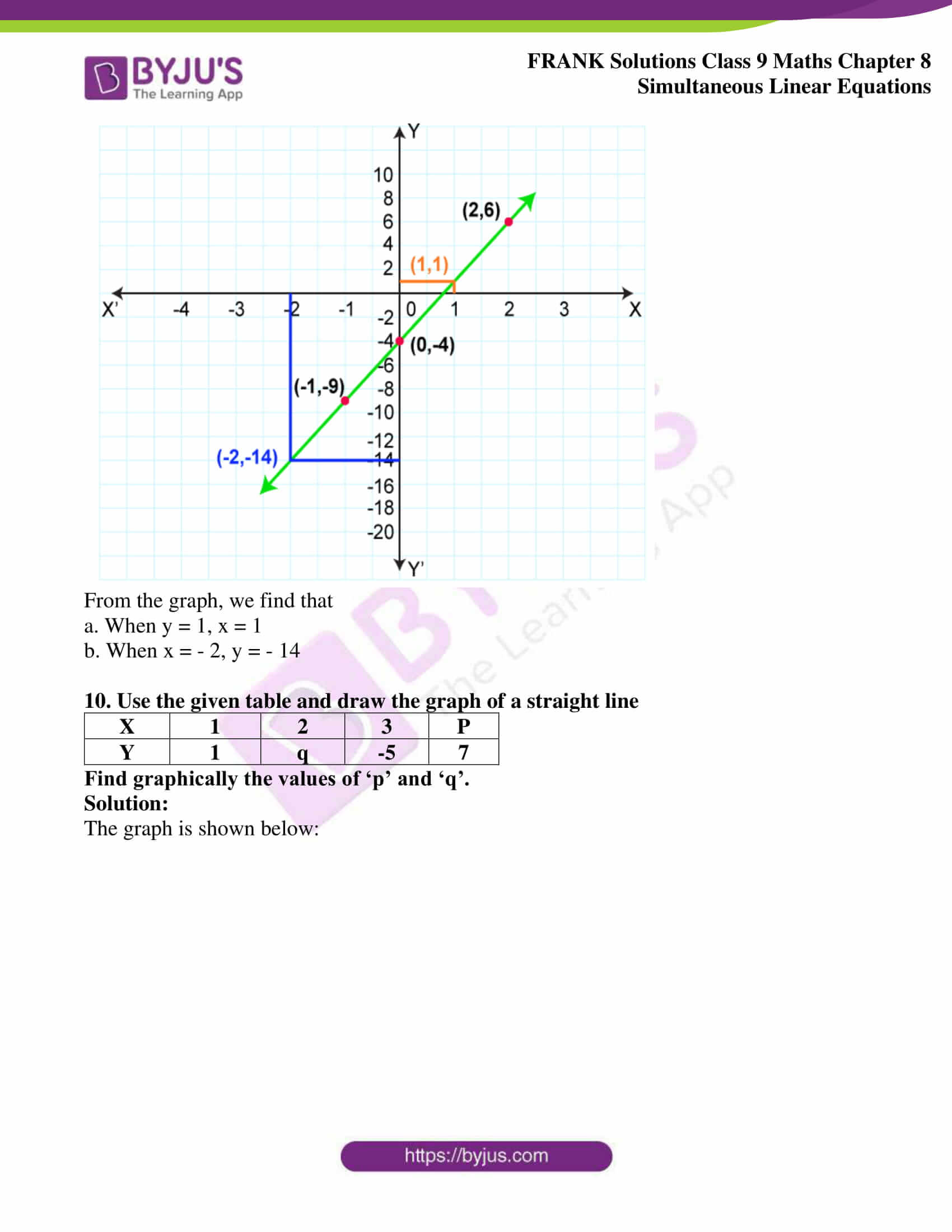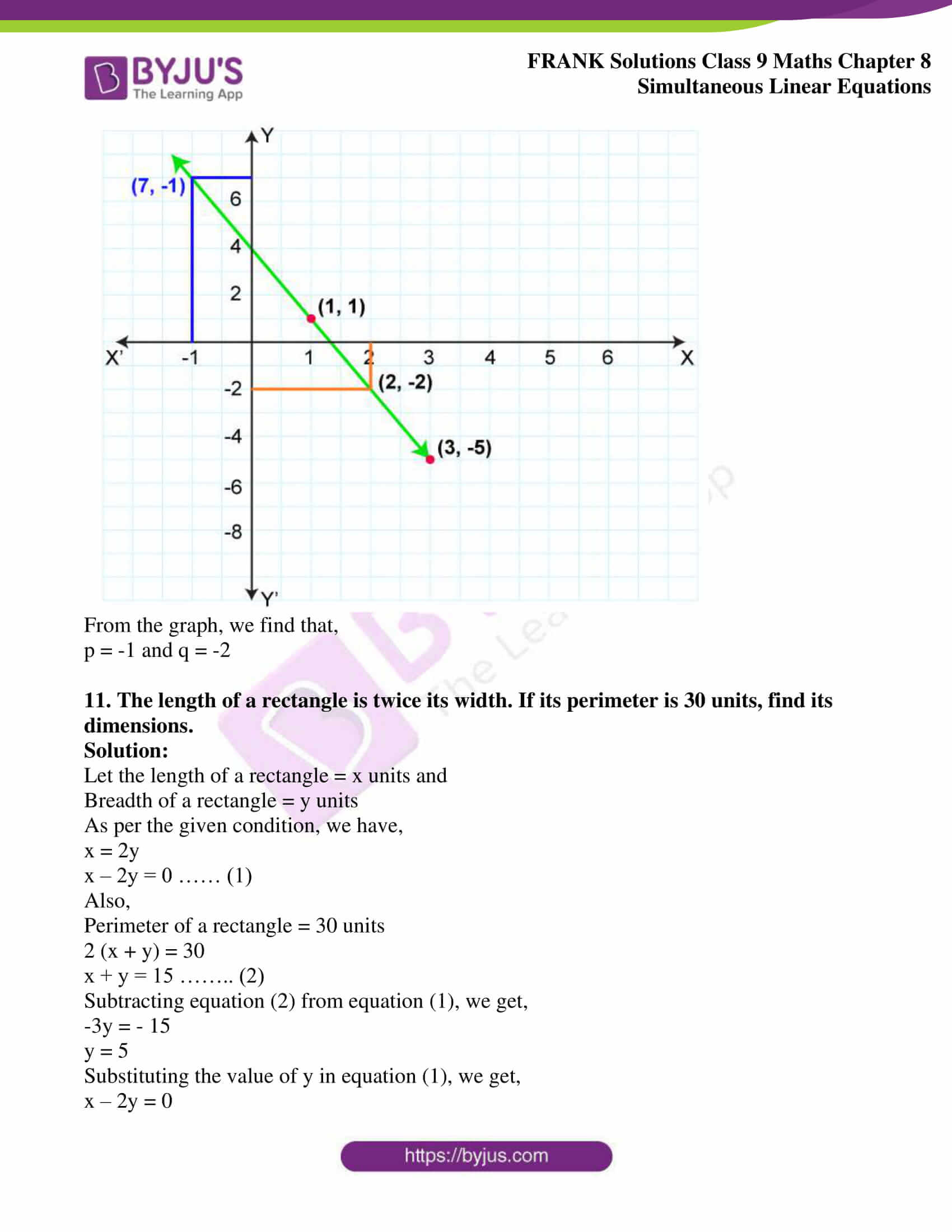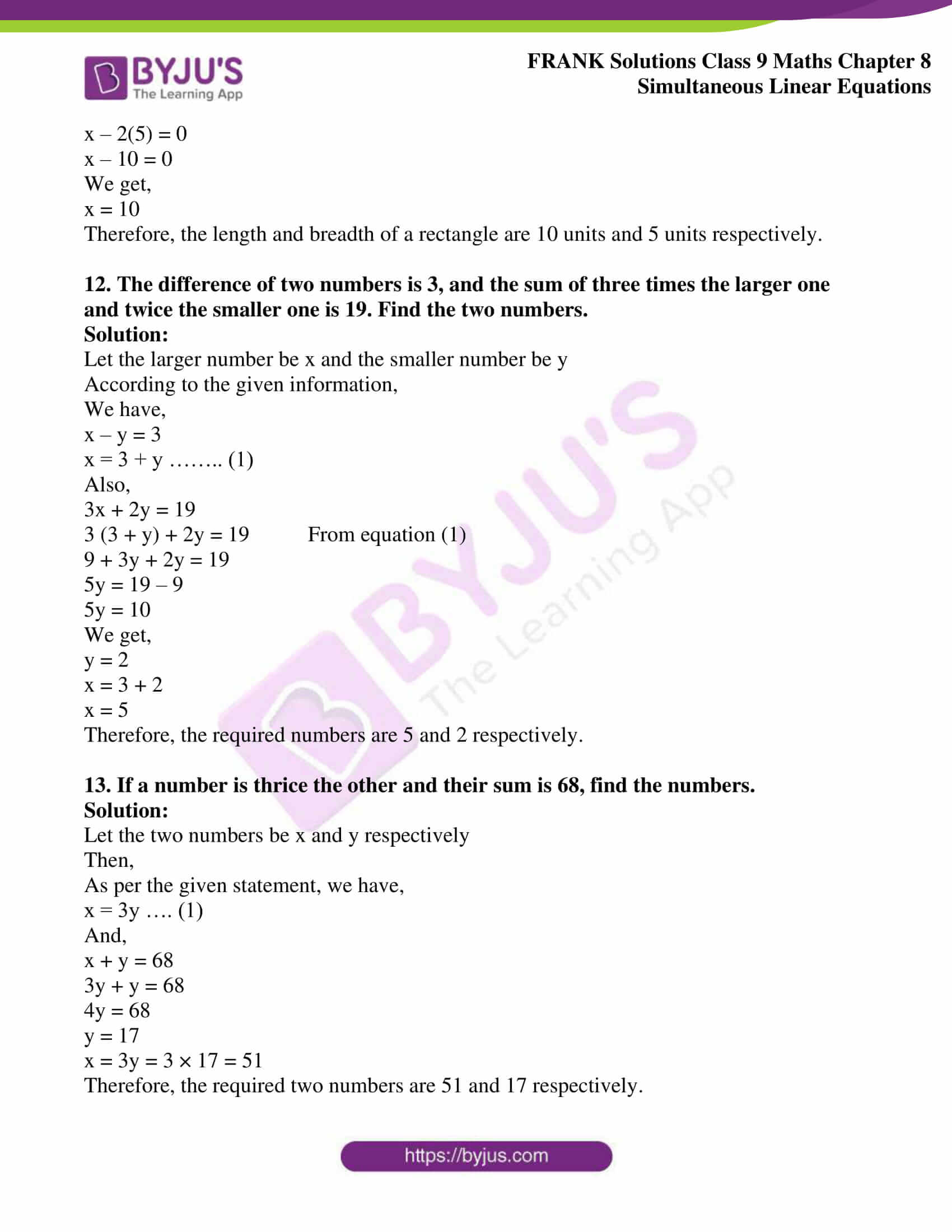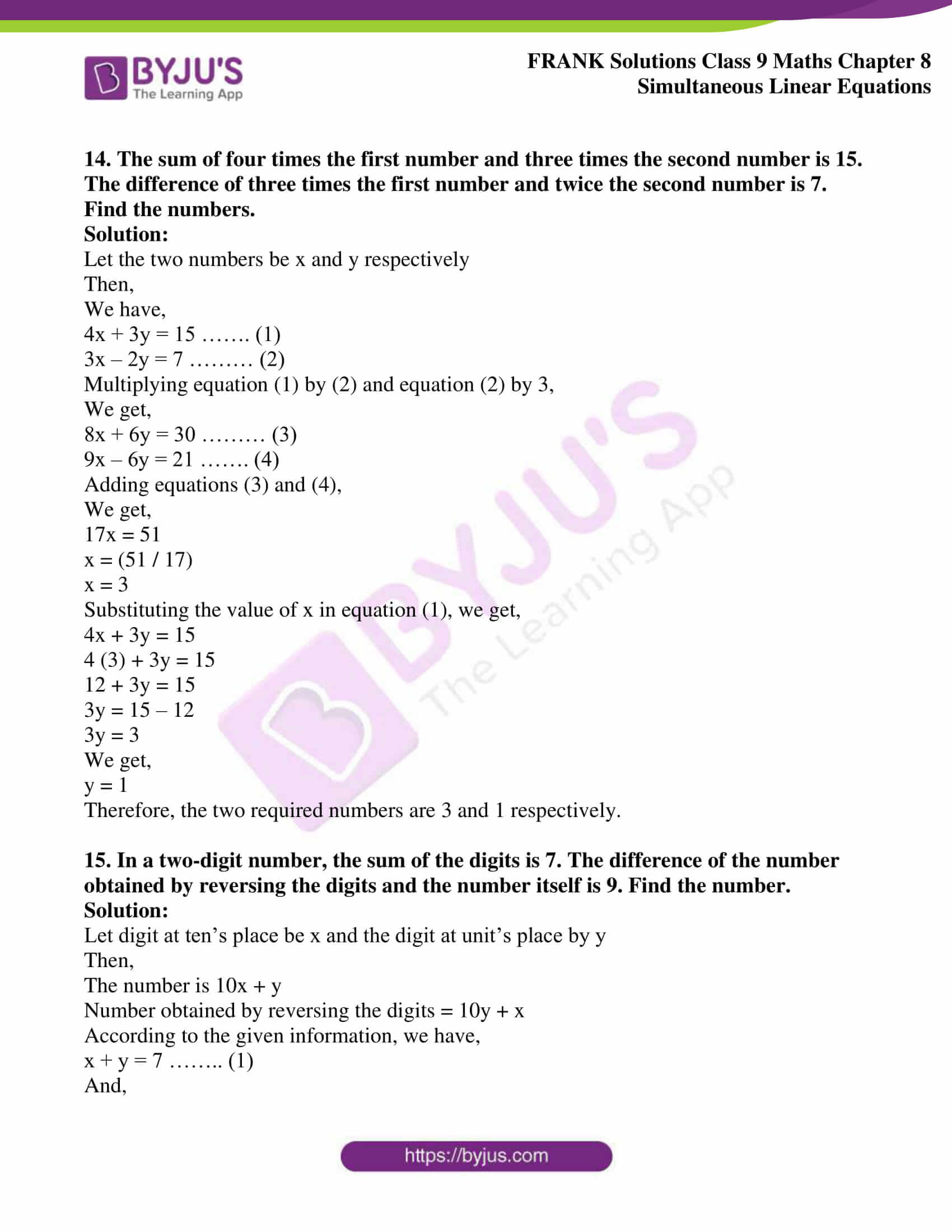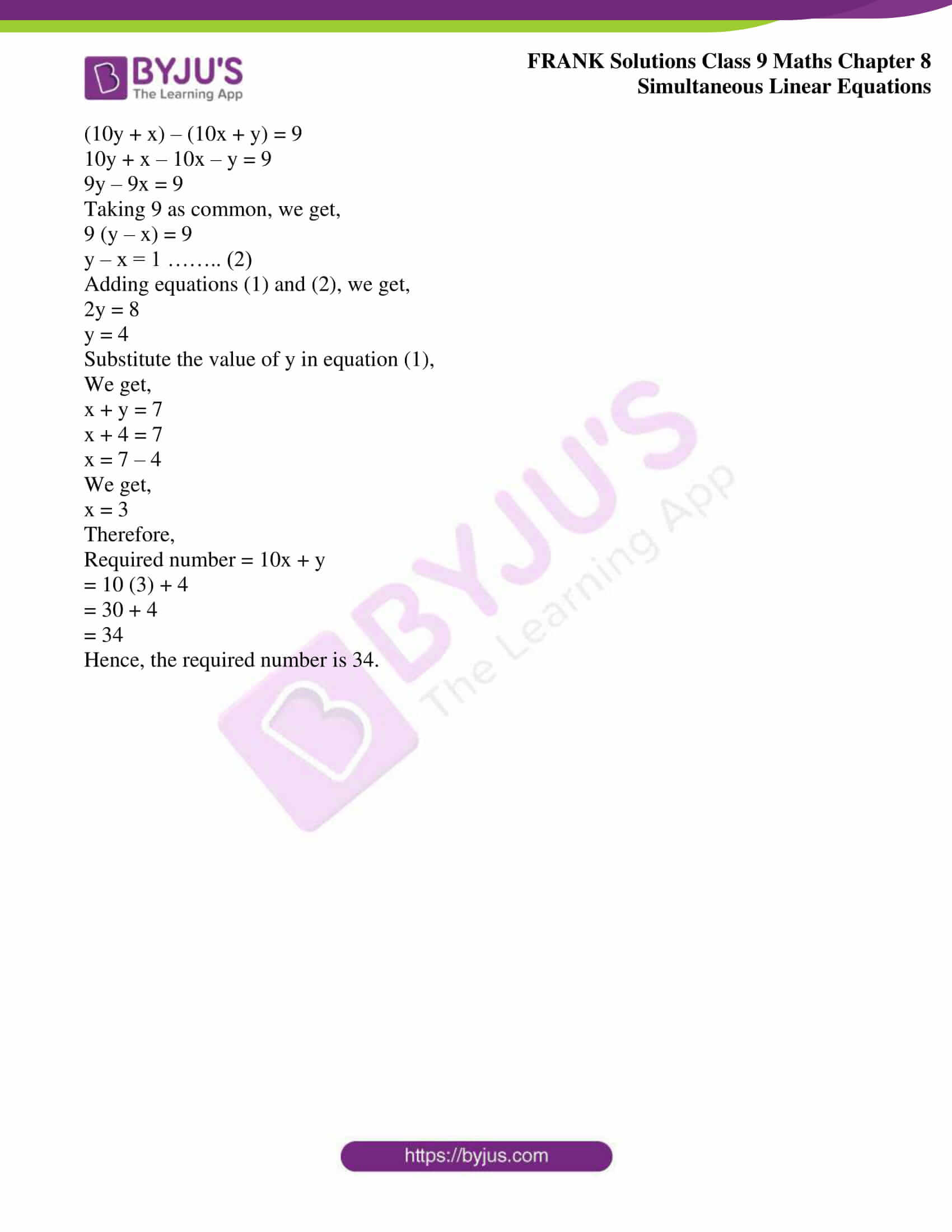## Access Frank Solutions for Class 9 Maths Chapter 8 Simultaneous Linear Equations

1. Solve the following simultaneous equations by the substitution method:

(a)2x + y = 8

3y = 3 + 4x

(b) x + 3y = 5

7x – 8y = 6

(c) 5x + 4y – 23 = 0

x + 9 = 6y

(d) 2x + 3y = 31

5x – 4 = 3y

(e) 7x – 3y = 31

9x – 5y = 41

(f) 13 + 2y = 9x

3y = 7x

(g) 0.5x + 0.7y = 0.74

0.3x + 0.5y = 0.5

(h) 0.4x + 0.3y = 1.7

0.7x – 0.2y = 0.8

(i) 3 – (x + 5) = y + 2

2 (x + y) = 10 + 2y

(j) 7 (y + 3) – 2 (x + 2) = 14

4 (y – 2) + 3 (x – 3) = 2

Solution:

Given

2x + y = 8 ……… (1)

3y = 3 + 4x ……… (2)

Now,

Consider the equation 2x + y = 8

y = 8 – 2x ……… (3)

Substituting the value of y in equation (2), we get,

3 (8 – 2x) = 3 + 4x

24 – 6x = 3 + 4x

– 6x – 4x = 3 – 24

– 10x = – 21

We get,

x = (21 / 10)

Now,

Putting the value of x in equation (3), we get,

y = 8 – 2x

y = 8 – 2 (21 / 10)

On further calculation, we get,

y = 8 – (21 / 5)

y = {(40 – 21) / 5}

We get,

y = (19 / 5)

Therefore, the solution set is {(21 / 10), (19 / 5)}

(b) Given

x + 3y = 5 ……… (1)

7x – 8y = 6 ……… (2)

Now,

Consider the equation x + 3y = 5

x = 5 – 3y ………… (3)

Substituting the value of x in equation (2), we get,

7 (5 – 3y) – 8y = 6

35 – 21y – 8y = 6

35 – 29y = 6

– 29y = 6 – 35

– 29y = – 29

We get,

y = 1

Putting the value of y in equation (3), we get,

x = 5 – 3y

x = 5 – 3(1)

x = 5 – 3

x = 2

Therefore, the solution set is (2, 1)

(c) Given

5x + 4y – 23 = 0 ………… (1)

x + 9 = 6y …………. (2)

Now,

Consider the equation (2),

x + 9 = 6y

x = 6y – 9 ………… (3)

Substituting the value of x in equation (1), we get,

5 (6y – 9) + 4y – 23 = 0

30y – 45 + 4y – 23 = 0

34 y – 68 = 0

34y = 68

y = (68 / 34)

We get,

y = 2

Putting the value of y in equation (3), we get,

x = 6 (2) – 9

x = 12 – 9

We get,

x = 3

Therefore, the solution set is (3, 2)

(d) Given

2x + 3y = 31 …….. (1)

5x – 4 = 3y …….. (2)

Now,

Consider the equation (1),

2x + 3y = 31

2x = 31 – 3y

x = {(31 – 3y) / 2} ………… (3)

Substituting the value of x in equation (2), we get,

5 {(31 – 3y) / 2} – 4 = 3y

{(155 – 15y) / 2} – 4 = 3y

On taking LCM, we get,

{(155 – 15y – 8) / 2} = 3y

147 – 15y = 6y

We get,

21y = 147

y = (147 / 21)

y = 7

Now,

Putting the value of y in equation (3), we get,

x = [{31 – 3(7)} / 2]

x = (31 – 21) / 2

x = (10 / 2)

We get,

x = 5

Therefore, the solution set is (5, 7)

(e) Given

7x – 3y = 31 ……… (1)

9x – 5y = 41 ………. (2)

Now,

Consider the equation (1),

7x – 3y = 31

7x = 31 + 3y

x = {(31 + 3y) / 7} …….. (3)

Substituting the value of x in equation (2), we get,

{9 (31 + 3y) / 7} – 5y = 41

{(279 + 27y) / 7} – 5y = 41

On taking LCM, we get,

(279 + 27y – 35y) / 7 = 41

279 – 8y = 287

– 8y = 287 – 279

– 8y = 8

We get,

y = – 1

Putting the value of y in equation (3), we get,

x = [{31 + 3(-1)} / 7]

x = (31 – 3) / 7

x = (28 / 7)

We get,

x = 4

Therefore, the solution set is (4, -1)

(f) Given

13 + 2y = 9x …… (1)

3y = 7x ……… (2)

Now,

Consider the equation (2),

3y = 7x

y = (7x / 3) ……… (3)

Substituting the value of y in equation (1), we get,

13 + 2 (7 / 3) x = 9x

13 + (14 / 3) x = 9x

9x – (14 / 3) x = 13

On taking LCM, we get,

{(27x – 14x) / 3} = 13

13x = 39

x = (39 / 13)

We get,

x = 3

Putting the value of x in equation (3), we get,

y = (7 / 3) x

y = (7 / 3) 3

We get,

y = 7

Therefore, the solution set is (3, 7)

(g) Given

0.5x + 0.7y = 0.74 ….. (1)

0.3x + 0.5y = 0.5 ……. (2)

Now,

Consider equation 0.5x + 0.7y = 0.74

0.5x = 0.74 – 0.7y

x = {(0.74 – 0.7y) / 0.5} …….. (3)

Substituting the value of x in equation (2), we get,

0.3 {(0.74 – 0.7y) / 0.5} + 0.5y = 0.5

{(0.222 – 0.21y) / 0.5} + 0.5y = 0.5

On taking LCM, we get,

{(0.222 – 0.21y + 0.25y) / 0.5} = 0.5

0.222 + 0.04y = 0.25

0.04y = 0.028

y = (0.028 / 0.04)

y = (28 / 40)

y = (7 / 10)

We get,

y = 0.7

Putting the value of y in equation (3), we get,

x = [{0.74 – 0.7 (0.7)} / 0.5]

x = (0.74 – 0.49) / 0.5

x = (0.25 / 0.5)

x = (25 / 50)

x = (1 / 2)

We get,

x = 0.5

Therefore, the solution set is (0.5, 0.7)

(h) Given

0.4x + 0.3y = 1.7 …….. (1)

0.7x – 0.2y = 0.8 ……. (2)

Multiplying both the equations by 10, we get,

4x + 3y = 17 ……. (3)

7x – 2y = 8 …….. (4)

Now,

Consider the equation,

4x + 3y = 17

4x = 17 – 3y

x = (17 – 3y) / 4 ………. (5)

Substituting the value of x in equation (4)

7 {(17 – 3y) / 4} – 2y = 8

{119 – 21y) / 4} – 2y = 8

On taking LCM, we get,

{(119 – 21y – 8y) / 4 = 8

119 – 29y = 32

-29y = 32 – 119

-29y = – 87

y = (- 87 / – 29)

We get,

y = 3

Putting the value of y in equation (5), we get,

x = [{17 – 3(3)} / 4]

x = (17 – 9) / 4

x = (8 / 4)

We get,

x = 2

Therefore, the solution set is (2, 3)

(i) Given

3 – (x + 5) = y + 2 ………. (1)

2 (x + y) = 10 + 2y ……… (2)

Now,

Consider equation (1)

3 – (x + 5) = y + 2

3 – x – 5 = y + 2

– x – 2 = y + 2

x + y = – 4

x = – 4 – y ………. (3)

Now,

Consider equation

2 (x + y) = 10 + 2y

2x + 2y = 10 + 2y

2x = 10

We get,

x = 5

Substituting the value of x in equation (3), we get,

5 = – 4 – y

y = – 4 – 5

y = – 9

Therefore, the solution set is (5, – 9)

(j) Given

7 (y + 3) – 2 (x + 2) = 14 ……. (1)

4 (y – 2) + 3 (x – 3) = 2 ………(2)

Consider the equation (1)

7 (y + 3) – 2 (x + 2) = 14

7y + 21 – 2x – 4 = 14

– 2x + 7y = 14 + 4 – 21

– 2x + 7y = -3

2x – 7y = 3

2x = 7y + 3

We get,

x = {(7y + 3) / 2} ……. (3)

Now,

Consider the equation 4 (y – 2) + 3(x – 3) = 2

4y – 8 + 3x – 9 = 2

3x + 4y = 19

Substituting the value of x, we get,

3 {(7y + 3) / 2} + 4y = 19 from (3)

(21y + 9) / 2} + 4y = 19

On taking LCM, we get,

(21y + 9 + 8y) / 2 = 19

29y + 9 = 38

29y = 29

We get,

y = 1

Substituting the value of y in equation (3), we get,

x = {(7y + 3) / 2}

x = {7(1) + 3} / 2

We get,

x = (10 / 2)

x = 5

Therefore, the solution set is (5, 1)

2. Solve the following simultaneous equations:

(i) 6x + 3y = 7xy

3x + 9y = 11xy

(ii) 8v – 3u = 5uv

6v – 5u = – 2uv

(iii) 3 (2u + v) = 7uv

3 (u + 3v) = 11uv

(iv) 2 (3u – v) = 5uv

2 (u + 3v) = 5uv

Solution:

(i) 6x + 3y = 7xy

3x + 9y = 11xy

Dividing both sides of each equation by xy, we get,

(6 / y) + (3 / x) = 7 …….. (1)

(3 / y) + (9 / x) = 11 ……. (2)

Multiplying equation (2) by 2, we get,

(6 / y) + (18 / x) = 22 ……… (3)

Subtracting (1) from (3), we get,

(15 / x) = 15

x = 1

Hence,

(3 / y) + 9 = 11

(3 / y) = 11 – 9

(3 / y) = 2

We get,

y = (3 / 2)

Therefore, the solution set is {1, (3 / 2)}

(ii) 8v – 3u = 5uv

6v – 5u = – 2uv

Dividing both sides of each equation by uv, we get,

(8 / u) – (3 / v) = 5 ……… (1)

(6 / u) – (5 / v) = – 2 …… (2)

Multiplying equation (1) by 3 and equation (2) by 4,

We get,

(24 / u) – (9 / v) = 15 ……… (3)

(24 / u) – (20 / v) = – 8 ……… (4)

Subtracting (4) from (3), we get,

(11 / v) = 23

v = (11 / 23)

Hence,

(6 / u) – (5 / 11) × 23 = – 2

(6 / u) – (115 / 11) = – 2

(6 / u) = – 2 + (115 / 11)

(6 / u) = (-22 + 115) / 11

(6 / u) = (93 / 11)

u = (6 × 11) / 93

We get,

u = (22 / 31)

Therefore, the solution set is {(22 / 31), (11 / 23)}

(iii) 3 (2u + v) = 7uv

3 (u + 3v) = 11uv

Dividing by uv, we get,

(6 / v) + (3 / u) = 7 ……… (1)

Consider,

3 (u + 3v) = 11uv

3u + 9v = 11uv

Dividing by uv, we get,

(3 / v) + (9 / u) = 11 …….. (2)

Multiplying (1) by 3, we get,

(18 / v) + (9 / u) = 21 …….. (3)

Subtracting (2) from (3), we get,

(15 / v) = 10

v = (15 / 10)

v = (3 / 2)

Hence,

(3 / u) = 7 – (6 / v) from (1)

(3 / u) = 7 – {(6 × (2 / 3)}

(3 / u) = 7 – 4

(3 / u) = 3

We get,

u = 1

Therefore, the solution set is {1, (3 / 2)}

(iv) 2 (3u – v) = 5uv

2 (u + 3v) = 5uv

2 (3u – v) = 5uv

6u – 2v = 5uv

Dividing by uv, we get,

(6 / v) – (2 / u) = 5 ……. (1)

2 (u + 3v) = 5uv

2u + 6v = 5uv

Dividing by uv, we get,

(2 / v) + (6 / u) = 5 …….. (2)

Multiplying equation (1) by 3, we get,

(18 / v) – (6 / u) = 15 ………… (3)

Adding equation (2) and (3), we get,

(20 / v) = 20

v = 1

Hence,

(6 / u) = 5 – (2 / 1)

(6 / u) = 3

u = (6 / 3)

We get,

u = 2

Therefore, the solution set is (2, 1)

3. Solve the following simultaneous equations:

(a) 13a – 11b = 70

11a – 13b = 74

(b) 41x + 53y = 135

53x + 41y = 147

(c) 65x – 33y = 97

33x – 65y = 1

(d) 103a + 51b = 617

97a + 49b = 583

Solution:

(a) The given equations are,

13a – 11b = 70 …….. (1)

11a – 13b = 74 …….. (2)

Multiplying equation (1) by 13 and equation (2) by 11, we get,

169a – 143b = 910 …….. (3)

121a – 143b = 814 ……… (4)

Subtracting equation (4) from equation (3), we get,

48a = 96

We get,

a = 2

Substituting the value of a in equation (1), we get,

13(2) – 11b = 70

26 – 11b = 70

– 11b = 70 – 26

– 11b = 44

We get,

b = – 4

Therefore, the solution set is (2, – 4)

(b) The given equations are,

41x + 53y = 135 …….. (1)

53x + 41y = 147 ……… (2)

Multiplying equation (1) by 53 and equation (2) by 41, we get,

2173x + 2809y = 7155 …… (3)

2173x + 1681y = 6027 …….. (4)

Subtracting equation (4) from (3) we get,

1128y = 1128

y = 1

Substituting the value of y in equation (1), we get,

41x + 53(1) = 135

41x + 53 = 135

41x = 135 – 53

41x = 82

We get,

x = 2

Therefore, the solution set is (2, 1)

(c) The given equations are,

65x – 33y = 97 ……. (1)

33x – 65y =1 …….. (2)

Multiplying equation (1) by 33 and equation (2) by 65, we get,

2145x – 1089y = 3201 ……. (3)

2145x – 4225y = 65 ……. (4)

Subtracting equation (4) from equation (3), we get,

3136y = 3136

y = 1

Substituting the value of y in equation (2), we get,

33x – 65 (1) = 1

33x – 65 = 1

33x = 1 + 65

33x = 66

We get,

x = 2

Therefore, the solution set is (2, 1)

(d) The given equations are

103a + 51b = 617 …… (1)

97a + 49b = 583 ……… (2)

Subtracting equation (2) from (1), we get,

6a + 2b = 34

Dividing throughout by 2, we get,

3a + b = 17 …… (3)

Adding both the equations, we get,

200a + 100b = 1200

Dividing throughout by 100, we get,

2a + b = 12 ….. (4)

Subtracting equation (4) from equation (3), we get,

a = 5

Substituting the value of a in equation (3), we get,

3(5) + b = 17

15 + b = 17

b = 17 – 15

b = 2

Therefore, the solution set is (5, 2)

4. Solve the following pairs of equations:

(a) (3 / 5) x – (2 / 3) y + 1 = 0

(1 / 3) y + (2 / 5) x = 4

(b) (x / 3) + (y / 4) = 11

(5x / 6) – (y / 3) = – 7

(c) (3 / 2x) + (2 / 3y) = 5

(5 / x) – (3 / y) = 1

(d) (3 / x) – (1 / y) = – 9

(2 / x) + (3 / y) = 5

(e) y – x = 0.8

[13 / {2 (x + y)}] = 1

(f) (2 / x) + (3 / y) = (9 / xy)

(4 / x) + (9 / y) = (21 / xy)

Where x ≠ 0, y ≠ 0

(g) (x + y) / xy = 2

(x – y) / xy = 6

(h) {2 / (x + 1)} – {1 / (y – 1)} = (1 / 2)

{1 / (x + 1)} + (2 / (y – 1)} = (5 / 2)

(i) {6 / (x + y)} = {7 / (x – y)} + 3

1 / {2 (x + y)} = 1 / {3 (x – y)}

Where x + y ≠ 0 and x – y ≠ 0

(j) {5 / (x + y)} – {2 / (x – y)} = -1

{15 / (x + y)} + {7 / (x – y)} = 10

(k) {2 / (3x + 2y)} + {3 / (3x – 2y)} = (17 / 5)

{5 / (3x + 2y)} + {1 / (3x – 2y)} = 2

(l) {xy / (x + y)} = (6 / 5)

{xy / (y – x)} = 6

where x + y ≠ 0 and y – x ≠ 0

Solution:

(a) Given equations are,

(3 / 5) x – (2 / 3) y + 1 = 0

(1 / 3) y + (2 / 5) x = 4

(3 / 5) x – (2 / 3) y + 1 = 0

On taking LCM, we get,

9x – 10y + 15 = 0

9x – 10y = – 15 ………. (1)

(1 / 3) y + (2 / 5) x = 4

On taking LCM, we get,

5y + 6x = 60

6x + 5y = 60 ……… (2)

Multiplying equation (2) by 2, we get,

12x + 10y = 120 …… (3)

Adding equations (1) and (3), we get,

21x = 105

x = 5

Substituting the value of x in equation (2), we get,

6 (5) + 5y = 60

30 + 5y = 60

5y = 60 – 30

5y = 30

y = 6

Therefore, the solution set is (5, 6)

(b) Given equations are,

(x / 3) + (y / 4) = 11

(5x / 6) – (y / 3) = – 7

(x / 3) + (y / 4) = 11

On taking LCM, we get,

4x + 3y = 132 ………. (1)

(5x / 6) – (y / 3) = – 7

On taking LCM, we get,

5x – 2y = – 42 …….. (2)

Multiplying equation (1) by 2 and equation (2) by 3, we get,

8x + 6y = 264 ……. (3)

15x – 6y = – 126 ….. (4)

Adding equations (3) and (4), we get,

23x = 138

x = 6

Substituting the value of x in equation (1), we get,

4(6) + 3y = 132

24 + 3y = 132

3y = 132 – 24

3y = 108

We get,

y = 36

Therefore, the solution set is (6, 36)

(c) Given equations are,

(3 / 2x) + (2 / 3y) = 5

(5 / x) – (3 / y) = 1

Let (1 / x) = a and (1 / y) = b

Then,

We have

(3 / 2) a + (2 / 3) b = 5

On taking LCM, we get,

9a + 4b = 30 ………. (1) and

5a – 3b = 1 ………… (2)

Multiplying equation (1) by 3 and equation (2) by 4, we get,

27a + 12b = 90 …….. (3)

20a – 12b = 4 ………. (4)

Adding equations (3) and (4), we get,

47a = 94

a = 2

Hence,

(1 / x) = 2

x = (1 / 2)

Substituting the value of a in (1), we get,

9 (2) + 4b = 30

18 + 4b = 30

4b = 30 – 18

4b = 12

We get,

b = 3

(1 / y) = 3

y = (1 / 3)

Therefore, the solution set is {(1 / 2), (1 / 3)}

(d) Given equations are,

(3 / x) – (1 / y) = – 9

(2 / x) + (3 / y) = 5

Let (1 / x) = a and (1 / y) = b

Then,

We have,

3a – b = – 9 ……. (1)

2a + 3b = 5 ……. (2)

Multiplying equation (1) by 3, we get,

9a – 3b = – 27 …….. (3)

Adding equations (2) and (3), we get,

11a = – 22

a = – 2

(1 / x) = – 2

x = (- 1 / 2)

Substituting the value of a in equation (1), we get,

3 (-2) – b = – 9

– 6 – b = – 9

b = – 6 + 9

b = 3

(1 / y) = 3

y = (1 / 3)

Therefore, the solution set is {(- 1 / 2), (1 / 3)}

(e) Given equations are,

y – x = 0.8

[13 / {2 (x + y)}] = 1

y – x = 0.8

– x + y = 0.8 ……… (1)

And [13 / {2 (x + y)}] = 1

On cross multiplication, we get,

13 = 2 (x + y)

13 = 2x + 2y

2x + 2y = 13 ……… (2)

Multiplying equation (1) by 2, we get,

– 2x + 2y = 1.6 …. .. (3)

Adding equations (2) and (3) we get,

4y = 14.6

y = 3.65

Substituting the value of y in equation (1), we get,

– x + 3.65 = 0.8

– x = – 2.85

x = 2.85

Therefore, the solution set is (2.85, 3.65)

(f) Given equations are,

(2 / x) + (3 / y) = (9 / xy)

(4 / x) + (9 / y) = (21 / xy)

Let (1 / x) = a and (1 / y) = b

Then,

We have,

2a + 3b = 9ab ……. (1)

4a + 9b = 21ab ….. (2)

Multiplying equation (1) by 2, we get,

4a + 6b = 18ab …………. (3)

Subtracting equation (3) from equation (2), we get,

3b = 3ab

a = 1

(1 / x) = 1

x = 1

Substituting the value of a in equation (1), we get,

2(1) + 3b = 9(1) b

2 + 3b = 9b

6b = 2

b = (1 / 3)

(1 / y) = (1 / 3)

y = 3

Therefore, the solution set is (1, 3)

(g) Given equations are,

(x + y) / xy = 2

(x – y) / xy = 6

(x + y) / xy = 2

x + y = 2xy …….. (1)

(x – y) / xy = 6

x – y = 6xy ……. (2)

Adding equations (1) and (2), we get,

2x = 8xy

y = (1 / 4)

Substituting the value of y in equation (1), we get,

x + (1 / 4) = 2x (1 / 4)

{(4x + 1) / 4} = (x / 2)

On cross multiplication, we get,

8x + 2 = 4x

4x = – 2

x = (- 1 / 2)

Therefore, the solution set is {(- 1 / 2), (1 / 4)}

(h) Given equations are,

{2 / (x + 1)} – {1 / (y – 1)} = (1 / 2)

{1 / (x + 1)} + (2 / (y – 1)} = (5 / 2)

Let {1 / (x + 1)} = a and {1 / (y – 1)} = b

Then,

We have,

2a – b = (1 / 2) ……….. (1)

a + 2b = (5 / 2) …….. (2)

Multiplying equation (1) by 2, we get,

4a – 2b = 1 ……… (3)

Adding equations (2) and (3), we get,

5a = (7 / 2)

a = (7 / 10)

{1 / (x + 1)} = (7 / 10)

On cross multiplication, we get,

10 = 7x + 7

7x = 3

x = (3 / 7)

Substituting the value of a in equation (3), we get,

4(7 / 10) – 2b = 1

(14 / 5) – 2b = 1

2b = (14 / 5) – 1

2b = (9 / 5)

b = (9 / 10)

{1 / (y – 1)} = (9 / 10)

On cross multiplication, we get,

10 = 9y – 9

9y = 19

y = (19 / 9)

Therefore, the solution set is {(3 / 7), (19 / 9)}

(i) Given equations are,

{6 / (x + y)} = {7 / (x – y)} + 3

1 / {2 (x + y)} = 1 / {3 (x – y)}

Let {1 / (x + y)} = a and {1 / (x – y)} = b

Then,

We have,

6a = 7b + 3

6a – 7b = 3 ……… (1)

And (1 / 2) a = (1 / 3) b

3a = 2b

Multiplying by 2, we get,

6a = 4b ….. (2)

Substituting the value of 6a in equation (1), we get,

4b – 7b = 3

– 3b = 3

b = -1

6a = – 4

a = (- 2 / 3)

x + y = (- 3 / 2) and x – y = -1

Adding both these equations, we get,

2x = (- 5 / 2)

x = (- 5 / 4)

(- 5 / 4) – y = – 1

y = (- 5 / 4) + 1

y = (- 1 / 4)

Therefore, the solution set is {(- 5 / 4), (- 1 / 4)}

(j) Given equations are,

{5 / (x + y)} – {2 / (x – y)} = -1

{15 / (x + y)} + {7 / (x – y)} = 10

Let {1 / (x + y)} = a and {1 / (x – y)} = b

Then,

We have,

5a – 2b = -1 ……… (1)

15a + 7b = 10 …….. (2)

Multiplying equation (1) by 3, we get,

15a – 6b = – 3 ……. (3)

Subtracting equation (3) from equation (2), we get,

13b = 13

b = 1

Substituting the value of b in equation (1), we get,

5a – 2(1) = – 1

5a = – 1 + 2

5a = 1

a = (1 / 5)

x + y = 5 and x – y = 1

Adding these two equations, we get,

2x = 6

x = 3

3 + y = 5

y = 5 – 3

y = 2

Therefore, the solution set is (3, 2)

(k) Given equations are,

{2 / (3x + 2y)} + {3 / (3x – 2y)} = (17 / 5)

{5 / (3x + 2y)} + {1 / (3x – 2y)} = 2

Let {1 / (3x + 2y)} = a and {1 / (3x – 2y)} = b

Then,

We have,

2a + 3b = (17 / 5) ……. (1)

5a + b = 2 ……. (2)

Multiplying equation (1) by 5 and equation (2) by 2, we get,

10a + 15b = 17 …….. (3)

10a + 2b = 4 …….. (4)

Subtracting equation (4) from equation (3), we get,

13b = 13

b = 1

Substituting the value of b in equation (2), we get,

5a + 1 = 2

5a = 1

a = (1 / 5)

3x + 2y = 5 and 3x – 2y = 1

Adding these two equations, we get,

6x = 6

x = 1

3(1) + 2y = 5

2y = 5 – 3

2y = 2

y = 1

Therefore, the solution set is (1, 1)

(l) Given equations are,

{xy / (x + y)} = (6 / 5)

{xy / (y – x)} = 6

{xy / (x + y)} = (6 / 5)

(x + y) / xy = (5 / 6)

(1 / y) + (1 / x ) = (5 / 6) …….. (1)

{xy / (y – x)} = 6

(y – x) / xy = (1 / 6)

(1 / x) – (1 / y) = (1 / 6) ………. (2)

Adding equations (1) and (2), we get,

(2 / x) = 1

x = 2

(1 / y) + (1 / 2) = (5 / 6)

(1 / y) = (5/ 6) – (1 / 2)

(1 / y) = (5 – 3) / 6

(1 / y) = (2 / 6)

(1 / y) = (1 / 3)

y = 3

Therefore, the solution set is (2, 3)

5. If 2x + y = 23 and 4x – y = 19; find the value of x – 3y and 5y – 2x

Solution:

Given

2x + y = 23 …….. (1)

4x – y = 19 ……… (2)

Adding equations (1) and (2), we get,

6x = 42

x = 7

Substitute the value of x in equation (1), we get,

2x + y = 23

2 (7) + y = 23

14 + y = 23

y = 23 – 14

y = 9

Hence,

x – 3y = 7 – 3 (9) = 7 – 27 = – 20

5y – 2x = 5 (9) – 2 (7)

= 45 – 14

= 31

6. Draw the graphs of the following linear equations:

(a) x = 3

(b) y + 5 = 0

(c) 3x + 2y – 6 = 0

(d) 5x – 5y = 8

Solution:

The graph of x = 3 is shown below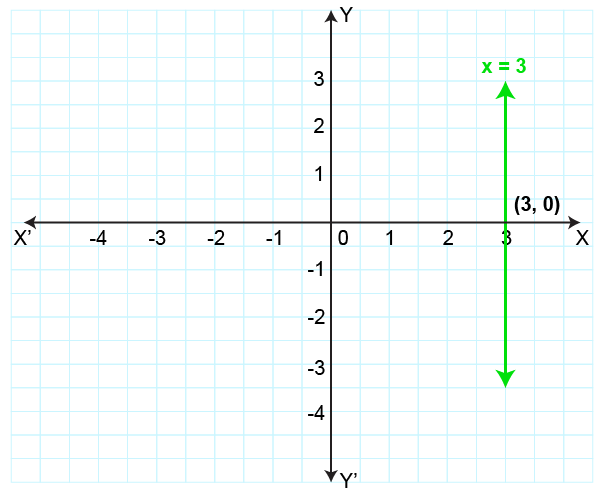(b) Given equation is,

y + 5 = 0

i.e, y = – 5

The graph is shown below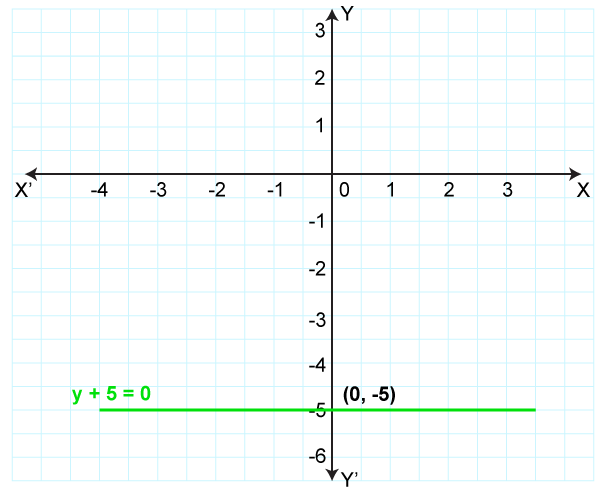(c) 3x + 2y – 6 = 0

3x + 2y = 6

2y = 6 – 3x

We get,

y = (6 – 3x) / 2

Corresponding values of x and y can be tabulated as follows:

 X 0 1 -1 Y 3 1.5 4.5

Plotting the points (0, 3), (1, 1.5) and (-1, 4.5),

We get the graph as shown below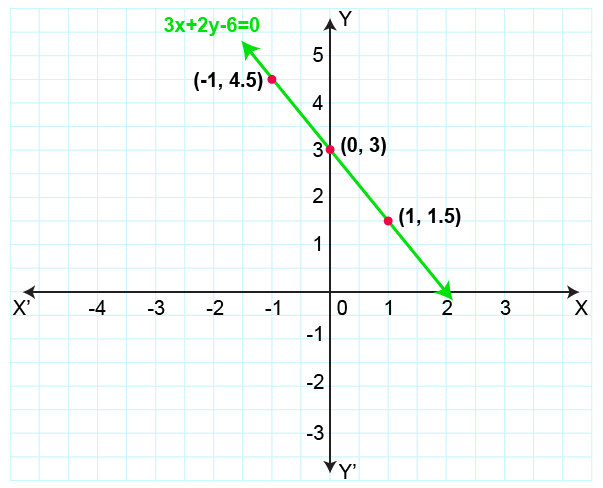(d) Given equation is,

5x – 5y = 8

5x = 8 – 5y

x = (8 – 5y) / 5

Corresponding values of x and y can be tabulated as follows:

 X 1.6 0.6 2.6 Y 0 1 -1

Plotting the points (1.6, 0), (0.6, 1) and (2.6, – 1),

We get the graph as shown below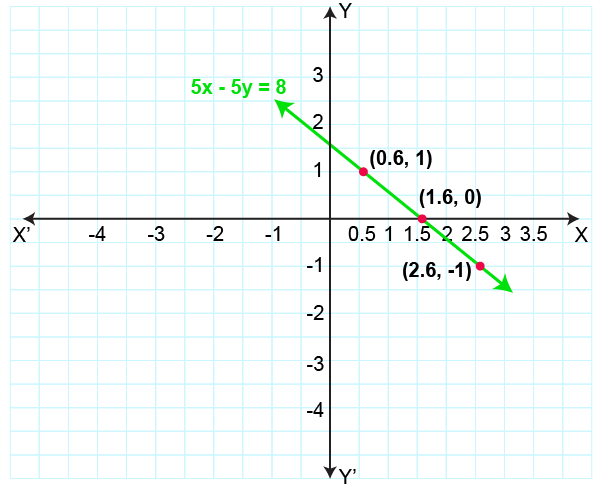7. Draw the graph for each of the following equation: Also, find the coordinates of the points where the graph of the equation meets the coordinate axes:

(a) (1 / 2) x + (1 / 3) y = 1

(b) {(3x + 14) / 2} = {(y – 10) / 5}

Solution:

Given

(1 / 2) x + (1 / 3) y = 1

3x + 2y = 6

2y = 6 – 3x

We get,

y = {(6 – 3x) / 2}

Corresponding values of x and y can be tabulated as follows:

 X 0 1 -1 Y 3 1.5 4.5

Plotting the points (0, 3), (1, 1.5) and (-1, 4.5),

We get the graph as shown below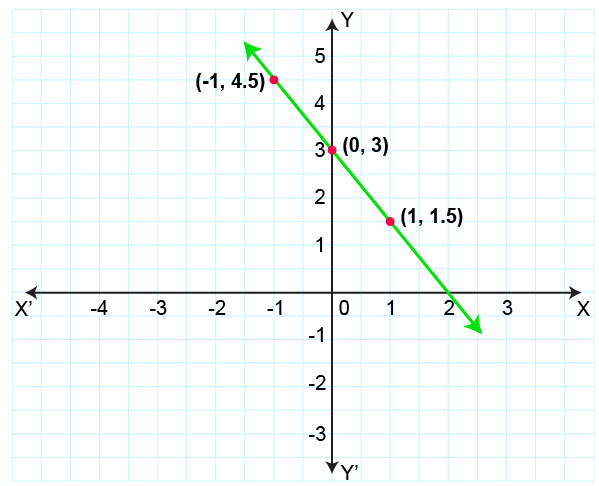Therefore, the graph of the equation meets the X – axis at (2, 0) and Y- axis at (0, 3)

(b) Given

{(3x + 14) / 2} = {(y – 10) / 5}

On cross multiplication, we get,

15x + 70 = 2y – 20

15x – 2y = – 20 – 70

15x – 2y = – 90

2y = 90 + 15x

y = {(90 + 15x) / 2}

Corresponding values of x and y can be tabulated as below

 X – 5 – 2 – 1 Y 7.5 30 37.5

Plotting the points (-5, 7.5), (-2, 30) and (-1, 37.5),

We get the graph as shown below: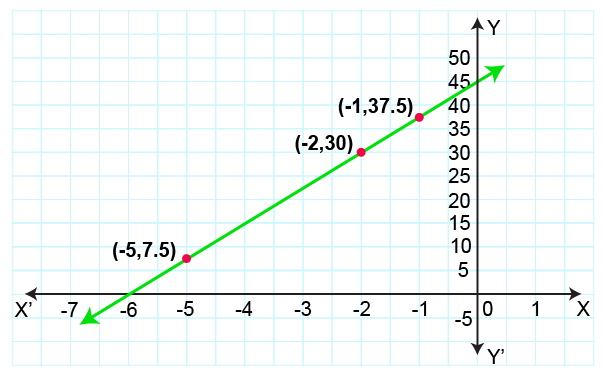Therefore, the graph of the equation meets the X-axis at (-6, 0) and Y-axis at (0, 45)

8. Draw the graph of the equation 4x – 3y + 12 = 0

Also, find the area of the triangle formed by the line drawn and the coordinate axes.

Solution:

Given equation is,

4x – 3y + 12 = 0

4x = 3y – 12

x = (3y – 12) / 4

Corresponding values of x and y can be tabulated as follows:

 X -3 – 1.5 0 Y 0 2 4

Plotting the points (-3, 0), (-1.5, 2) and (0, 4)

We get the graph as shown below: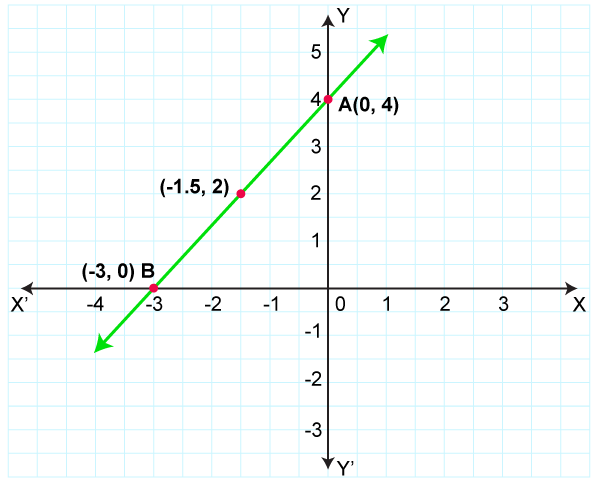Hence,

Area of △OAB = (1 / 2) × OB × OA

Area of △OAB = (1 / 2) × 3 × 4

We get,

Area of △OAB = 6 sq. units

9. Draw the graph of the equation

y = 5x – 4 Find graphically

a. the value of x, when y = 1

b. the value of y, when x = – 2

Solution:

Given equation is,

y = 5x – 4

Corresponding values of x and y can be tabulated as follows:

 X 0 2 -1 Y – 4 6 -9

Plotting the points (0, -4), (2, 6) and (-1, -9),

We get the graph as shown below: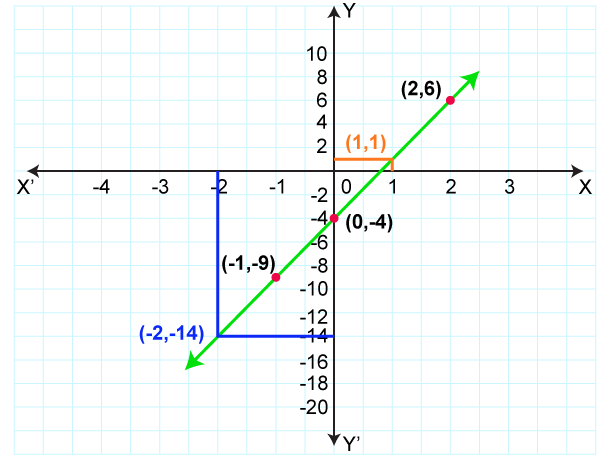From the graph, we find that

a. When y = 1, x = 1

b. When x = – 2, y = – 14

10. Use the given table and draw the graph of a straight line

 X 1 2 3 P Y 1 q -5 7

Find graphically the values of ‘p’ and ‘q’.

Solution:

The graph is shown below: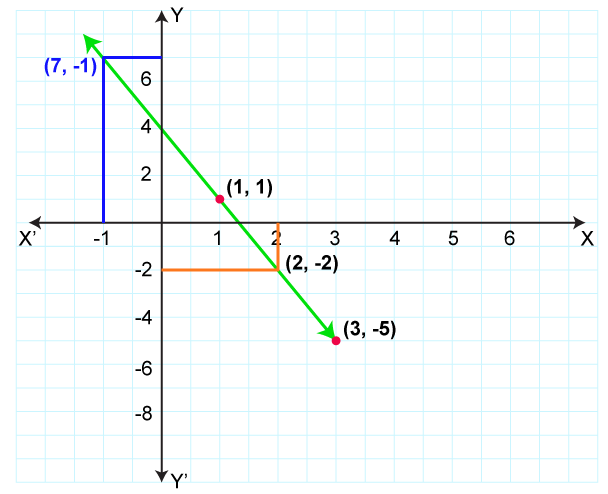From the graph, we find that,

p = -1 and q = -2

11. The length of a rectangle is twice its width. If its perimeter is 30 units, find its dimensions.

Solution:

Let the length of a rectangle = x units and

Breadth of a rectangle = y units

As per the given condition, we have,

x = 2y

x – 2y = 0 …… (1)

Also,

Perimeter of a rectangle = 30 units

2 (x + y) = 30

x + y = 15 …….. (2)

Subtracting equation (2) from equation (1), we get,

-3y = – 15

y = 5

Substituting the value of y in equation (1), we get,

x – 2y = 0

x – 2(5) = 0

x – 10 = 0

We get,

x = 10

Therefore, the length and breadth of a rectangle are 10 units and 5 units respectively.

12. The difference of two numbers is 3, and the sum of three times the larger one and twice the smaller one is 19. Find the two numbers.

Solution:

Let the larger number be x and the smaller number be y

According to the given information,

We have,

x – y = 3

x = 3 + y …….. (1)

Also,

3x + 2y = 19

3 (3 + y) + 2y = 19 From equation (1)

9 + 3y + 2y = 19

5y = 19 – 9

5y = 10

We get,

y = 2

x = 3 + 2

x = 5

Therefore, the required numbers are 5 and 2 respectively.

13. If a number is thrice the other and their sum is 68, find the numbers.

Solution:

Let the two numbers be x and y respectively

Then,

As per the given statement, we have,

x = 3y …. (1)

And,

x + y = 68

3y + y = 68

4y = 68

y = 17

x = 3y = 3 × 17 = 51

Therefore, the required two numbers are 51 and 17 respectively.

14. The sum of four times the first number and three times the second number is 15. The difference of three times the first number and twice the second number is 7. Find the numbers.

Solution:

Let the two numbers be x and y respectively

Then,

We have,

4x + 3y = 15 ……. (1)

3x – 2y = 7 ……… (2)

Multiplying equation (1) by (2) and equation (2) by 3,

We get,

8x + 6y = 30 ……… (3)

9x – 6y = 21 ……. (4)

We get,

17x = 51

x = (51 / 17)

x = 3

Substituting the value of x in equation (1), we get,

4x + 3y = 15

4 (3) + 3y = 15

12 + 3y = 15

3y = 15 – 12

3y = 3

We get,

y = 1

Therefore, the two required numbers are 3 and 1 respectively.

15. In a two-digit number, the sum of the digits is 7. The difference of the number obtained by reversing the digits and the number itself is 9. Find the number.

Solution:

Let digit at ten’s place be x and the digit at unit’s place by y

Then,

The number is 10x + y

Number obtained by reversing the digits = 10y + x

According to the given information, we have,

x + y = 7 …….. (1)

And,

(10y + x) – (10x + y) = 9

10y + x – 10x – y = 9

9y – 9x = 9

Taking 9 as common, we get,

9 (y – x) = 9

y – x = 1 …….. (2)

Adding equations (1) and (2), we get,

2y = 8

y = 4

Substitute the value of y in equation (1),

We get,

x + y = 7

x + 4 = 7

x = 7 – 4

We get,

x = 3

Therefore,

Required number = 10x + y

= 10 (3) + 4

= 30 + 4

= 34

Hence, the required number is 34.